# Motion in One Dimension

## Exercise 2(A)

#### Question 1

Differentiate between scalar and vector quantities, giving two examples of each.

Differences between scalar and vector quantities are as follows —

Scalar QuantityVector Quantity
These are physical quantities which are expressed only by their magnitude.These physical quantities require magnitude as well as the direction to express them, then only their meaning is complete.
We need two parameters to express a scalar quantity.We require three parameters to express a vector quantity.
The parameters are:
(i) unit in which the quantity is being measured
(ii) numerical value of the measured quantity.
The parameters are:
(i) unit
(ii) direction
(iii) numerical value of quantity.
Scalar quantities can be added, subtracted, multiplied and divided by simple arithmetic methods.Vector quantities follow different algebra for their addition, subtraction, multiplication and division.
Example:
(i) If we say, mass of a body is 5 kg, it has a complete meaning and we are completely expressing the mass of the body.
(ii) mass, length, time, etc.
Example:
(i) A body is displaced by 5 metre towards east, then it has a complete meaning.
(ii) velocity, acceleration, force, etc.

#### Question 2

State whether the following quantity is a scalar or vector?

(a) Pressure

(b) Force

(c) Momentum

(d) Energy

(e) Weight

(f) Speed

(a) Pressure — Scalar quantity

(b) Force — Vector quantity

(c) Momentum — Vector quantity

(d) Energy — Scalar quantity

(e) Weight — Vector quantity

(f) Speed — Scalar quantity

#### Question 3

When is a body said to be at rest?

A body is said to be at rest if it does not change its position with respect to its immediate surroundings.

Example — When we are sitting on a platform and we see a tree nearby, we say the tree is at rest because it does not change its position with respect to us.

#### Question 4

When is a body said to be in motion ?

A body is said to be in motion if it changes its position with respect to its immediate surroundings.

Example — When we are sitting on a platform and we see a train leaving the station, we say that the train is in motion because it is continuously changing its position with respect to us.

#### Question 5

What do you mean by motion in one direction ?

When a body moves along a straight line path, its motion is said to be one dimensional motion.

Example — A stone falling down vertically or a motion of a train on a straight track etc., are one dimensional (or rectilinear motion).

#### Question 6

Define displacement. State its unit.

Displacement is vector quantity. It's magnitude is the shortest distance from the initial to the final position of the body and it's direction is from the initial position to the final position.

Unit — The S.I. unit of displacement is metre (m) and C.G.S. unit is centimetre (cm)

#### Question 7

Differentiate between distance and displacement.

The difference between distance and displacement is as follows:

DistanceDisplacement
It is the length of the path traversed by the object in a certain time.It is the distance travelled by the object in a specified direction in a certain time (i.e. it is the shortest distance between the final and the initial positions).
It is a scalar quantity i.e., it has only the magnitude.It is a vector quantity i.e., it has both magnitude and direction.
It depends on the path followed by the object.It does not depend on the path followed by the object.
It is always positive.It can be positive or negative depending on its direction.
It can be more than or equal to the magnitude of displacement.Its magnitude can be less than or equal to the distance, but can never be greater than the distance.
It may not be zero even if displacement is zero, but it can not be zero if displacement is not zero.It is zero if distance is zero, but it can be zero if distance is not zero.

#### Question 8

Can displacement be zero even if distance is not zero? Give one example to explain your answer.

Yes, the displacement can be zero, even if the distance is not zero.

If a body, after travelling, comes back to its starting point, the displacement is zero but the distance travelled is not zero.

Example — When a body is thrown vertically upwards from a point A on the ground, after some time it comes back to the same point A, then the displacement of the body is zero but the distance travelled by the body is not zero.

#### Question 9

When is the magnitude of displacement equal to the distance ?

The magnitude of displacement is equal to the distance, when the motion is in a fixed direction.

#### Question 10

Define velocity. State its unit.

The velocity of a body is the distance travelled per second by the body in a specified direction.

It is a vector quantity.

$\text{S.I. unit of velocity} = \dfrac{\text{metre}}{\text{second}} = \dfrac{\text{m}}{\text{s}} \\[0.5em] \text{C.G.S. unit of velocity} = \dfrac{\text{centimetre}}{\text{second}} = \dfrac{\text{cm}}{\text{s}} \\[0.5em]$.

#### Question 11

Define speed. What is its S.I. unit ?

Speed of a body is the rate of change of distance with time .

It is a scalar quantity. It is generally represented by u or v .

If a body travels a distance S in time t, then its speed v is —

$\text{Speed (v)} = \dfrac{\text{Distance (S)}}{\text{Time (t)}} = \dfrac{\text{S}}{\text{t}} \\[0.5em]$

$\text{S.I. unit of speed} = \dfrac{\text{metre}}{\text{second}} = \dfrac{\text{m}}{\text{s}} \\[0.5em] \text{C.G.S. unit of speed} = \dfrac{\text{centimetre}}{\text{second}} = \dfrac{\text{cm}}{\text{s}} \\[0.5em]$.

#### Question 12

Distinguish between speed and velocity.

The difference between speed and velocity is as follows:

SpeedVelocity
The distance travelled per second by a moving object is called its speed.The distance travelled per second by a moving object in a particular direction is called its velocity.
It is a scalar quantity. The speed does not tell us the direction of motion.It is a vector quantity. The velocity tells us the speed as well as the direction of motion.
The speed is always positive since direction is not taken into consideration.The velocity can be positive or negative depending upon the direction of motion.
After one round in a circular path, the average speed is not zero.After completing each round in a circular path, the average velocity is zero.

#### Question 13

Which quantity speed or velocity gives the direction of motion of a body.

Velocity gives direction of motion of body as it is a vector quantity. Speed is a scalar quantity hence, it does not say anything about direction of motion.

#### Question 14

When is the instantaneous speed same as the average speed?

In case of a body moving with uniform speed, the instantaneous speed and the average speed are equal (same as the uniform speed) because, in case of uniform speed, neither the speed nor the direction change.

#### Question 15

Distinguish between uniform velocity and variable velocity.

The difference between uniform velocity and variable velocity is as follows:

Uniform VelocityVariable Velocity
(or non-uniform)
If a body travels equal distances in a particular direction, in equal intervals of time, the body is said to be moving with a uniform velocity.If a body moves unequal distances in a particular direction in equal intervals of time or it moves equal distances in equal intervals of time, but its direction of motion does not remain the same, then the velocity of the body is said to be variable (non uniform).
Example — A body, once started on a frictionless surface, moves with uniform velocity.Example — The motion of a body in circular path, even with uniform speed is with variable velocity as the direction of motion of body continuously changes with time.

#### Question 16

Distinguish between average speed and average velocity.

The difference between average speed and average velocity is as follows —

Average SpeedAverage Velocity
The ratio of the total distance travelled by the body to the total time of journey is called its average speed.If the velocity of a body moving in a particular direction changes with time, the ratio of displacement to the time taken in the entire journey is called its average velocity.
Avg Speed =
$\dfrac{\text{Total Distance}}{\text{Total time}}$
Avg Velocity =
$\dfrac{\text{Displacement}}{\text{Total time}}$
Average speed can never be zero.It can be zero, even if average speed is a non-zero value.

#### Question 17

Give an example of motion of a body moving with a constant speed, but with a variable velocity. Draw a diagram to represent such a motion.

The motion of a body in circular path, is an example of a body moving with a constant speed and variable velocity because the direction of motion of body changes continuously with time.

At any instant, the velocity is along the tangent to the circular path at that point.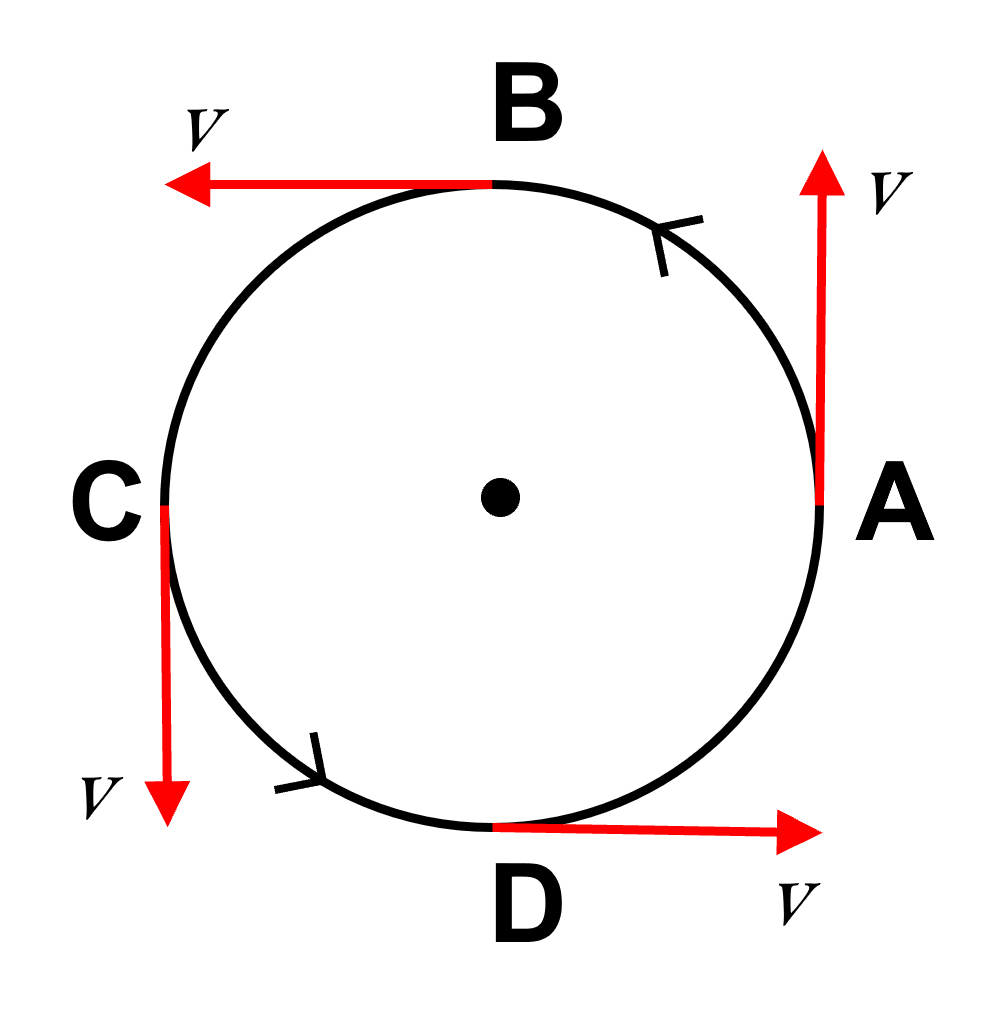The figure above shows the direction of velocity v at different points A, B, C and D of the circular path.

#### Question 18

Give an example of motion in which average speed is not zero, but average velocity is zero.

If a body starts its motion and comes back to the same point after a certain time, (e.g., in a circular motion,) the displacement is zero, so the average velocity is also zero, but the total distance travelled is not zero and therefore, the average speed is not zero.

#### Question 19

Define acceleration. State its S.I. unit.

Acceleration is the rate of change of velocity with time.

Numerically, acceleration is equal to the change in velocity in 1 s.

i.e.,

$\text{Acceleration} = \dfrac{\text{Change in velocity}}{\text{Time interval}} \\[0.5em] \text{S.I. unit of acceleration} = \dfrac{\text{meter}}{\text{second}^2} = \dfrac{\text{m}}{\text{s}^2}\\[0.5em] \text{C.G.S. unit of acceleration} = \dfrac{\text{centimeter}}{\text{second}^2} = \dfrac{\text{cm}}{\text{s}^2}\\[0.5em]$

#### Question 20

Distinguish between acceleration and retardation.

The differences between acceleration and retardation are as follows —

AccelerationRetardation
If the velocity of a body increases with time, it is called acceleration.If the velocity of a body decreases with time, it is called retardation.
As it is increase in velocity per second so it is positive acceleration.As it is decrease in velocity per second so retardation is negative acceleration.

#### Question 21

Differentiate between uniform acceleration and variable acceleration.

The differences between uniform acceleration and variable acceleration are as follows:

Uniform AccelerationVariable acceleration
The acceleration is said to be uniform (or constant) when equal changes in velocity take place in equal intervals of time.If changes in velocity are not same in the same intervals of time, the acceleration is said to be variable.
Example — The motion of a body under gravity (e.g., free fall of a body)Example — The motion of a vehicle on a crowded(or hilly) road.

#### Question 22

What is meant by the term retardation? Name its S.I. unit.

If the velocity of a body decreases with time, it is called retardation. As it is decrease in velocity per second, so, retardation is negative acceleration.

It's unit is same as that of acceleration.

$\text{S.I. unit of retardation} = \dfrac{\text{meter}}{\text{second}^2} = \dfrac{\text{m}}{\text{s}^2}\\[0.5em] \text{C.G.S. unit of retardation} = \dfrac{\text{centimeter}}{\text{second}^2} = \dfrac{\text{cm}}{\text{s}^2}\\[0.5em]$

#### Question 23

Which of the quantity, velocity or acceleration determines the direction of motion ?

Velocity determines the direction of motion as velocity is the distance travelled per second by a moving object in a particular direction.

Positive or negative sign of velocity indicates the direction of motion.

Acceleration is the rate of change of velocity with time. It does not say anything about direction of motion.

Positive or negative sign of acceleration tell us weather the velocity is increasing or decreasing.

#### Question 24

Give one example of each of the following:

(a) Uniform velocity

(b) Variable velocity

(c) Variable acceleration

(d) Uniform retardation

(a) Uniform velocity — The rain drops reach on earth’s surface falling with uniform velocity.

(b) Variable velocity — The motion of a freely falling body is with variable velocity because although the direction of motion of the body does not change, but the speed continuously increases.

(c) Variable acceleration — The motion of a vehicle on a hilly (or crowded) road.

(d) Uniform retardation — Motion of a vehicle, reaching a destination.

#### Question 25

The diagram below shows the pattern of the oil on the road, dripping at a constant rate from a moving car. What information do you get from it about the motion of car?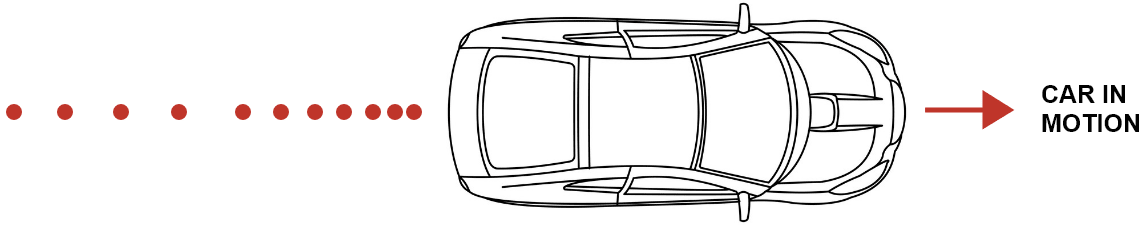With the help of the given diagram, we observe that the red dots (dripping oil) are initially at regular intervals and later the gap between the dots decreases, which implies that initially the car was covering equal distances in equal intervals of time but later the distance is decreasing.

Thus, we can infer that initially the car is moving with a constant speed and then it slows down.

#### Question 26

Define the term acceleration due to gravity. State its average value.

When a body falls freely under the gravity, the acceleration produced in the body due to earth's gravitational attraction is called acceleration due to gravity.

When a body falls down, its velocity increases with time, so the acceleration is +g, while if the body moves vertically upwards, its velocity decreases with time, so the acceleration is -g.

The value of g varies from place to place, so an average value is considered. The average value of ‘g’ is 9.8 ms-2 or nearly 10 ms-2.

#### Question 27

'The value of g remains same at all places on the earth surface'. Is this statement true? Give reason for your answer.

No, the value of 'g' is not same at all places on the earth's surface. The value of g varies from place to place so an average value is considered. The average value of 'g' is 9.8 ms-2 or nearly 10 ms-2. The value of g decreases with altitude and also with depth from the earth's surface.

The value of 'g' is maximum at the poles and minimum at the equator on the earth surface.

#### Question 28

If a stone and a pencil are dropped simultaneously in vacuum from the top of a tower, which of the two will reach the ground first ? Give reason.

Both will reach the ground simultaneously as value of g does not depend on the mass of the body. Acceleration due to gravity is same ( = g ) on both and there is no effect of friction and buoyancy due to air.

## Multiple Choice Type

#### Question 1

A vector quantity is —

1. Work
2. Pressure
3. Distance
4. Velocity ✓

A vector quantity has both magnitude and direction. Velocity has both magnitude and direction, so it is a vector quantity.

#### Question 2

The S.I. unit of velocity is —

1. Km h-1
2. m min-1
3. km min-1
4. m s-1

Velocity is the rate of change of displacement (m) of a body with time (s) so its unit is m s-1.

#### Question 3

The unit of retardation is —

1. m s-1
2. m s-2
3. m
4. m s-2

Retardation is decline in velocity (ms-1) of a body with time (s), so its unit is ms-2. Retardation is negative value of acceleration and has same unit as acceleration.

#### Question 4

A body when projected up with an initial velocity u goes to a maximum height h in time t and then comes back at the point of projection. The correct statement is —

1. The average velocity is 2h/t
2. The acceleration is zero
3. The final velocity on reaching the point of projection is 2u
4. The displacement is zero ✓

The displacement is zero because the initial and the final position of the body is same.

#### Question 5

18 km h-1 is equal to —

1. 10 m s-1
2. 5 m s-1
3. 18 m s-1
4. 1.8 m s-1

As,

$18 \text{kmh}^{-1} = 18 \dfrac{\text{km}}{\text{h}} = \dfrac {18 \times 1000 m}{60 \times 60 \text{s}} \\[0.5em] \Rightarrow 18 \text{kmh}^{-1} = \dfrac {18 \times 10 \text{m}}{6 \times 6 \text{s}} \\[0.5em] \Rightarrow 18 \text{\text{kmh}}^{-1}= \dfrac {5 \times \text{m}}{\text{s}} \\[0.5em]$

Hence, 18 km h-1 is equal to 5 m s-1.

## Numericals

#### Question 1

The speed of a car is 72 km h-1. Express it in m s-1.

As,

$72 \text {km h}^{-1} = \dfrac{72 \text {km}}{1 \text {h}} = \dfrac {72 \times 1000 \text {m}}{(60 \times 60) \text {s}} \\[0.5em] \Rightarrow 72 \text {km h}^{-1} = \dfrac {72 \times 10 \text {m}}{(6 \times 6 )\text {s}} \\[0.5em] \Rightarrow 72 \text {\text {km h}}^{-1} = \dfrac {20 \times \text {m}}{\text {s}} \\[0.5em]$

Hence, 72km h-1 is equal to 20 m s-1.

#### Question 2

Express 15 m s-1 in km h-1 .

As,

$15 \text {m s}^{-1} = 15 \dfrac{\text {m}}{\text {s}} = \dfrac {15 \times 60 \times 60 }{1000} \\[0.5em] \Rightarrow 15 \text {m s}^{-1} = \dfrac {15 \times 6 \times 6 }{10} \\[0.5em] \Rightarrow 15 \text {m s}^{-1}= 54 \text {km h}^{-1} \\[0.5em]$

Hence, 15 m s-1 is equal to 54 kmh-1.

#### Question 3

Express each of the following in m s -1

(a) 1 km h-1

(b) 18 km min-1

(a) As,

$1 \text{km h}^{-1} = \dfrac{1 \text{km}}{1\text{h}} = \dfrac {1 \times 1000 \text{m}}{60 \times 60 \text{s}} \\[0.5em] \Rightarrow 1 \text{km h}^{-1} = \dfrac {1 \times 10 \text{m}}{6 \times 6 \text{s}} \\[0.5em] \Rightarrow 1 \text{km h}^{-1} = \dfrac {0.278 \times \text{m}}{\text{s}} \\[0.5em]$

Hence, 1 km h-1 is equal to 0.278 ms-1.

(b) As,

$18 \text{km min}^{-1} = 18 \dfrac{\text{km}}{\text{min}} = \dfrac {18 \times 1000 \text{m}}{60 \text{s}} \\[0.5em] \Rightarrow 18 \text{kmh}^{-1} = \dfrac {18 \times 10 \text{m}}{6 \text{s}} \\[0.5em] \Rightarrow 18 \text{kmh}^{-1}= \dfrac {300 \times \text{ m}}{\text{s}} \\[0.5em]$

Hence, 18 km h-1 is equal to 300 m s-1.

#### Question 4

Arrange the following speeds in increasing order — 10 m s-1, 1 km min-1, 18 km h-1.

In order to arrange in increasing order of speeds, the units of the given speeds must be same.

Hence, we convert all the three in m s-1.

(a) 10 m s-1 = 10 m s-1   .... 
(already in m s-1)

(b) 1 km min-1,

$\text{1 km min}^{-1} = \dfrac{\text{1 km}}{\text{1 min}} = \dfrac {1 \times 1000 \text{m}}{ 60 \text{s}} \\[0.5em] \Rightarrow \text{1 km min}^{-1} = \dfrac {1 \times 100 \text{m}}{6\text{s}} \\[0.5em] \Rightarrow \text{1 km min}^{-1} = \dfrac {16.66 \times \text{m}}{\text{s}} \\[0.5em]$

Hence,
1 km min-1 = 16.66 m s-1   .... 

(c) 18 km h-1,

As,

$\text{18 km h}^{-1} = 18 \dfrac{km}{h} = \dfrac {18 \times 1000 m}{60 \times 60 s} \\[0.5em] \Rightarrow \text{18 km h}^{-1} = \dfrac {18 \times 10 m}{6 \times 6s} \\[0.5em] \Rightarrow \text{18 km h}^{-1} = \dfrac {5 \times m}{s} \\[0.5em]$

Hence,
18km h-1 = 5 m s-1   .... 

From 1, 2 and 3, we get the increasing order as follows —

18 km h-1, 10 m s-1, 1 km min-1.

#### Question 5

A train takes 3 h to travel from Agra to Delhi with a uniform speed of 65 km h-1. Find the distance between the two cities.

As we know,

Distance = Speed x Time

Given.

time = 3 h

speed = 65 km h-1

Substituting the values in the formula above, we get,

Distance = 65 x 3 = 195 km

Hence, the distance between the two cities = 195 km .

#### Question 6

A car travels first 30 km with a uniform speed of 60 km h-1 and then next 30 km with a uniform speed of 40 km h -1.

Calculate —

(i) the total time of journey,

(ii) the average speed of the car.

Given,

Distance travelled S1 = 30 km

Speed v1 = 60 km h-1

Distance travelled S2 = 30 km

Speed v2 = 40 km h-1

(i) As we know,

$\text {Time (t)} = \dfrac {\text {distance (S)}}{\text {speed (v)}}$

Substituting the values in the formula above, we get,

$\text{t}_1 = \dfrac{\text{S}_1}{\text{v}_1} \\[0.5em] \text{t}_1 = \dfrac{30 \text{km}}{60 \text{km h}^{-1}} \\[0.5em] \Rightarrow \text{t}_1 = \dfrac{1}{2} \text{h} \\[0.5em] \Rightarrow \text{t}_1 = 0.5 \text{h} \\[0.5em]$

Converting 0.5 h to min we get,

$\Rightarrow \text{t}_1 = 0.5 \times 60 \text{ min} \\[0.5em] \Rightarrow \text{t}_1 = 30 \text{ min} \\[0.5em]$

and

$\text{t}_2 = \dfrac{\text{S}_2}{\text{v}_2} \\[0.5em] \text{t}_2 = \dfrac{30 \text{km}}{40 \text{km h}^{-1}} \\[0.5em] \Rightarrow \text{t}_2 = \dfrac{3}{4} \text{h} \\[0.5em] \Rightarrow \text{t}_2 = 0.75 \text{h} \\[0.5em]$

Converting 0.75 h to min we get,

$\Rightarrow \text{t}_2 = 0.75 \times 60 \text{ min} \\[0.5em] \Rightarrow \text{t}_2 = 45 \text{ min} \\[0.5em]$

Total time t = t1 + t2
= 30 min + 45 min
= 75 min

Hence, Total time of journey = 75 min.

(ii) As we know,

Total distance S = S1 + S2
= 30 km + 30 km
= 60 km

Hence, Total distance travelled = 60 km.

As we know,

$\text{Average speed} = \dfrac{\text{total distance}}{\text{total time taken}} \\[0.5em] \text{Average speed} = \dfrac{60 \text { km}}{1.25 \text { h}} \\[0.5em] \Rightarrow \text{Average speed} = 48 \text { kmh} ^{-1}$

Hence, Average speed of the car = 48 kmh-1

#### Question 7

A train takes 2 h to reach station B from station A, and then 3 h to return from station B to station A. The distance between the two stations is 200 km.

Find —

(i) The average speed

(ii) The average velocity of the train

Given,

Distance travelled S1 = 200 km

time t1 = 2 h

Distance travelled S2 = 200 km

time t2 = 3 h

(i) As we know,

$\text{Average speed} = \dfrac{\text{total distance}}{\text{total time taken}} \\[0.5em]$

Total distance travelled S = S1 + S2
= 200 km + 200 km
= 400 km

Total time taken t = t1 + t2
= 2 h + 3 h
= 5 h

Substituting the values of total distance travelled and total time taken in the formula of Average Speed above, we get,

$\text{Average speed} = \dfrac{400 \text { km}}{5 \text { h}} \\[0.5em] \Rightarrow \text{Average speed} = 80 \text { kmh} ^{-1} \\[0.5em]$

Hence, Average speed of the car = 80 km h-1

(ii) Displacement = 0 (since final position is same as initial position)

Hence, Average velocity = 0 ( as displacement = 0)

#### Question 8

A car moving on a straight path covers a distance of 1 km due east in 100 s.

What is

(i) the speed and

(ii) the velocity of car ?

As we know,

$\text{Speed (v)} = \dfrac{\text{distance (S)}}{\text{time (t)}} \\[0.5em]$

Given,

Distance travelled S = 1 km = 1000 m,

time t = 100 s

Substituting the values in the formula above, we get,

$\text{Speed (v)} = \dfrac{1000 \text { m} }{ 100 \text{ s}} \\[0.5em] \Rightarrow \text{Speed (v)} = 10 \text { ms}^{-1} \\[0.5em]$

Hence, speed of the car is 10 m s-1

(ii) The magnitude of velocity of the car is same as that of speed of car i.e., 10 m s-1

However, when we talk about velocity, we mention direction also.

Hence, velocity of car is 10 m s-1 due east.

#### Question 9

A body starts from rest and acquires a velocity 10 m s -1 in 2 s. Find its acceleration.

Given,

Initial velocity (u) = 0

Final velocity (v) = 10 m s -1

Time (t) = 2 s

As we know,

$\text {a} = \dfrac {\text {final vel. (v)} - \text {initial vel. (u)}}{\text {time (t)}}$

Substituting the values in the formula above, we get,

$\text {Acceleration (a)} = \dfrac {10 - 0}{2} \\[0.5em] \text {Acceleration (a)} = \dfrac {10\text{ ms}^{-1}}{2 \text{ s}} \\[0.5em] \text {Acceleration (a)} = 5 \text {ms}^{-2} \\[0.5em]$

Hence, acceleration of the body is 5 m s -2.

#### Question 10

A car starting from rest acquires a velocity of 180 m s-1 in 0.05 h. Find the acceleration.

As we know,

$\text {a} = \dfrac {\text {final vel. (v)} - \text {initial vel. (u)}}{\text {time (t)}}$

Given,

Initial velocity (u) = 0

Final velocity (v) = 180 m s -1

Time (t) = 0.05 h

Converting 0.05 h to s we get,

$0.05 \text{h} = 0.05 \times 60 \times 60 \\[0.5em] 0.05 \text{h} = 0.05 \times 3600 \\[0.5em] 0.05 \text{h} = 180 \text {s} \\[0.5em]$

Substituting the values in the formula above, we get,

$\text {Acceleration (a)} = \dfrac {180 - 0}{180} \\[0.5em] \text {Acceleration (a)} = \dfrac {180 \text { ms}^{-1}}{180 \text { s}} \\[0.5em] \Rightarrow \text {Acceleration (a)} = 1 \text { ms}^{-2} \\[0.5em]$

Hence, acceleration of the body is 1 m s -2.

#### Question 11

A body is moving vertically upwards. Its velocity changes at a constant rate from 50 m s -1 to 20 m s -1 in 3 s . What is its acceleration ?

As we know,

$\text {a} = \dfrac {\text {final vel. (v)} - \text {initial vel. (u)}}{\text {time (t)}}$

Given,

Initial velocity (u) = 50 m s -1

Final velocity (v) = 20 m s -1

Time (t) = 3 s

Substituting the values in the formula above, we get,

$\text {Acceleration (a)} = \dfrac {20 - 50}{3} \\[0.5em] \text {Acceleration (a)} = - \dfrac {30\text { ms}^{-1}}{3\text { s}} \\[0.5em] \Rightarrow \text {Acceleration (a)} = -10 \text { ms}^{-2} \\[0.5em]$

Hence, acceleration of the body is -10 m s -2. Negative sign shows that the velocity decreases with time, so retardation is 10 m s -2.

#### Question 12

A toy car initially moving with a uniform velocity of 18 km h-1 comes to a stop in 2 s. Find the retardation of the car in S.I. units.

As we know,

$\text {a} = \dfrac {\text {final vel. (v)} - \text {initial vel. (u)}}{\text {time (t)}}$

Given,

Initial velocity (u) = 18 km h-1

To convert initial velocity u to m s-1

$18 \text { km h}^{-1} = \dfrac{18 \text { km}}{1 \text{ h}} = \dfrac {18 \times 1000 \text{ m}}{60 \times 60 \text{ s}} \\[0.5em] \Rightarrow 18 \text { km h}^{-1} = \dfrac {18 \times 10 \text{ m}}{6 \times 6 \text{ s}} \\[0.5em] \Rightarrow 18 \text { km h}^{-1} = 5 \text{ m s}^{-1} \\[0.5em]$

Hence, 18 km h-1 is equal to 5 m s-1.

Final velocity (v) = 0

Time (t) = 2 s

Substituting the values in the formula above, we get,

$\text {Acceleration (a)} = \dfrac {0 - 5}{2} \\[0.5em] \text {Acceleration (a)} = -\dfrac {5\text { ms}^{-1}}{2\text { s}} \\[0.5em] \Rightarrow \text {Acceleration (a)} = - 2.5 \text { ms}^{-2} \\[0.5em]$

Hence, acceleration of the body is -2.5 m s -2. Negative sign shows that the velocity decreases with time, so retardation is 2.5 m s -2.

#### Question 13

A car accelerates at a rate of 5 m s-2. Find the increase in its velocity in 2 s.

As we know,

$\text {a} = \dfrac {\text {final vel. (v)} - \text {initial vel. (u)}}{\text {time (t)}}$

Hence,

${\text {Velocity Increase}} = \text {Acceleration (a)} \times {\text{time(t)}}$

Given,

a = 5 m s-2

t = 2 s

Substituting the values in the formula above, we get,

$\text {Velocity Increase} = 5 \text{ m s}^{-2} \times 2 \text{ s} \\[0.5em] \Rightarrow \text {Velocity Increase} = 10 \text{ m s}^{-1} \\[0.5em]$

Hence, increase in velocity = 10 m s -1.

#### Question 14

A car is moving with a velocity 20 m s -1 . The brakes are applied to retard it at a rate of 2 m s -2. What will be the velocity after 5 s of applying the brakes ?

As we know,

$\text {a} = \dfrac {\text {final vel. (v)} - \text {initial vel. (u)}}{\text {time (t)}}$

and retardation is negative acceleration.

Hence,

$\text {final vel. (v)} - \text {initial vel. (u)} \\[0.5em] = \text {Retardation(-a)} \times {\text {time(t)}}$

Given,

a = - 2 m s-2

t = 5 s

u = 20 m s -1

Substituting the values in the formula above, we get,

$\text {final vel. (v)} - 20 \text{m s}^{-1} = - 2 \text{m s}^{-2} \times 5 s \\[0.5em] \text {v} - 20 \text{m s}^{-1} = - 10 \text{m s}^{-1} \\[0.5em] \Rightarrow \text {v} = (20 - 10) \text{m s}^{-1} \\[0.5em] \Rightarrow \text {v} = 10 \text{m s}^{-1} \\[0.5em]$

Hence, final velocity = 10 m s -1.

#### Question 15

A bicycle initially moving with a velocity 5.0 m s -1 accelerates for 5 s at a rate of 2 m s-2 . What will be its final velocity?

As we know,

$\text {a} = \dfrac {\text {final vel. (v)} - \text {initial vel. (u)}}{\text {time (t)}}$

Hence,

$\text {final vel. (v)} - \text {initial vel. (u)} \\[0.5em] = \text {Acceleration (a)} \times {\text {time (t)}}$

Given,

a = 2 m s-2

t = 5 s

u = 5.0 m s -1

Substituting the values in the formula above, we get,

$\text {final vel. (v)} - 5 \text{m s}^{-1} = 2 \text{m s}^{-2} \times 5 s \\[0.5em] \text {v} - 5 \text{m s}^{-1} = 10 \text{m s}^{-1} \\[0.5em] \Rightarrow \text {v} = (10 + 5) \text{m s}^{-1} \\[0.5em] \Rightarrow \text {v} = 15 \text{m s}^{-1} \\[0.5em]$

Hence, final velocity = 15 m s -1.

#### Question 16

A car is moving in a straight line with speed 18 km h-1. It is stopped in 5s by applying the brakes.

Find —

(i) the speed of car in m s -1,

(ii) the retardation and

(iii) the speed of car after 2 s of applying the brakes .

Given,

speed of car = 18 km h-1

(i) To convert speed to m s-1

$18 \text {km h}^{-1} = \dfrac{18 \text {km}}{1 \text{h}} = \dfrac {18 \times 1000 \text{m}}{60 \times 60 \text {s}} \\[0.5em] \Rightarrow 18 \text {km h}^{-1} = \dfrac {18 \times 10 \text {m}}{6 \times 6 \text {s}} \\[0.5em] \Rightarrow 18 \text {km h}^{-1} = 5 \text {m s}^{-1} \\[0.5em]$

Hence, 18 km h-1 is equal to 5 m s-1.

(ii) As the car is stopped, the final velocity = 0

initial velocity (u) = 18 km h-1

As we know,

$\text {a} = \dfrac {\text {final vel. (v)} - \text {initial vel. (u)}}{\text {time (t)}}$

Given,

Initial velocity (u) = 5 m s-1

Final velocity (v) = 0

Time (t) = 5 s

Substituting the values in the formula above, we get,

$\text {Acceleration (a)} = \dfrac {0 - 5}{5} \\[0.5em] \text {Acceleration (a)} = -\dfrac {5}{5} \\[0.5em] \Rightarrow \text {Acceleration (a)} = - 1 \text {ms}^{-2} \\[0.5em]$

Hence, acceleration of the car is -1 m s-2. Negative sign shows that the velocity decreases with time, so retardation is 1 m s -2.

(iii) Given,

t = 2 s

a = -1 m s-2

u = 5 m s-1

Substituting the values in the formula for acceleration, we get,

$- 1 \text {ms}^{-2} = \dfrac {(v - 5 ) \text {ms}^{-1}}{2 \text {s}} \\[0.5em] - 2 \text {ms}^{-1} = (v - 5) {ms}^{-1} \\[0.5em] \Rightarrow v = (5 - 2) \text {ms}^{-1} \\[0.5em] \Rightarrow v = 3 \text {ms}^{-1} \\[0.5em]$

Hence, the speed of car after 2 s of applying the brakes = 3 m s-1.

## Exercise 2(B)

#### Question 1

For the motion with uniform velocity, how is the distance travelled related to the time?

For the motion with uniform velocity, distance is directly proportional to time.

#### Question 2

What informations about the motion of a body are obtained from the displacement-time graph ?

In a displacement time graph, the time is on the X axis and the displacement of the body is on the Y axis. As, velocity is the ratio of displacement and time, therefore, the slope gives the velocity.

When slope is positive, it implies body is moving away from the starting (reference) point.

When slope is negative, it implies body is returning towards the starting (reference) point.

#### Question 3

(a) What does the slope of a displacement-time graph represent?

(b) Can displacement-time sketch be parallel to the displacement axis ? Give reason to your answer.

(a) As, velocity is the ratio of displacement and time, therefore, the slope gives the velocity.

When slope is positive, it implies body is moving away from the starting (reference) point.

When slope is negative, it implies body is returning towards the starting (reference) point.

(b) No, the displacement-time sketch can never be a straight line, parallel to the displacement axis because such a line would mean that the distance covered by the body in a certain direction increases without any increase in time (i.e., the velocity of the body is infinite) which is impossible.

#### Question 4

Draw a displacement-time graph for a boy going to school with a uniform velocity.

Below is the displacement-time graph for a boy going to school with a uniform velocity: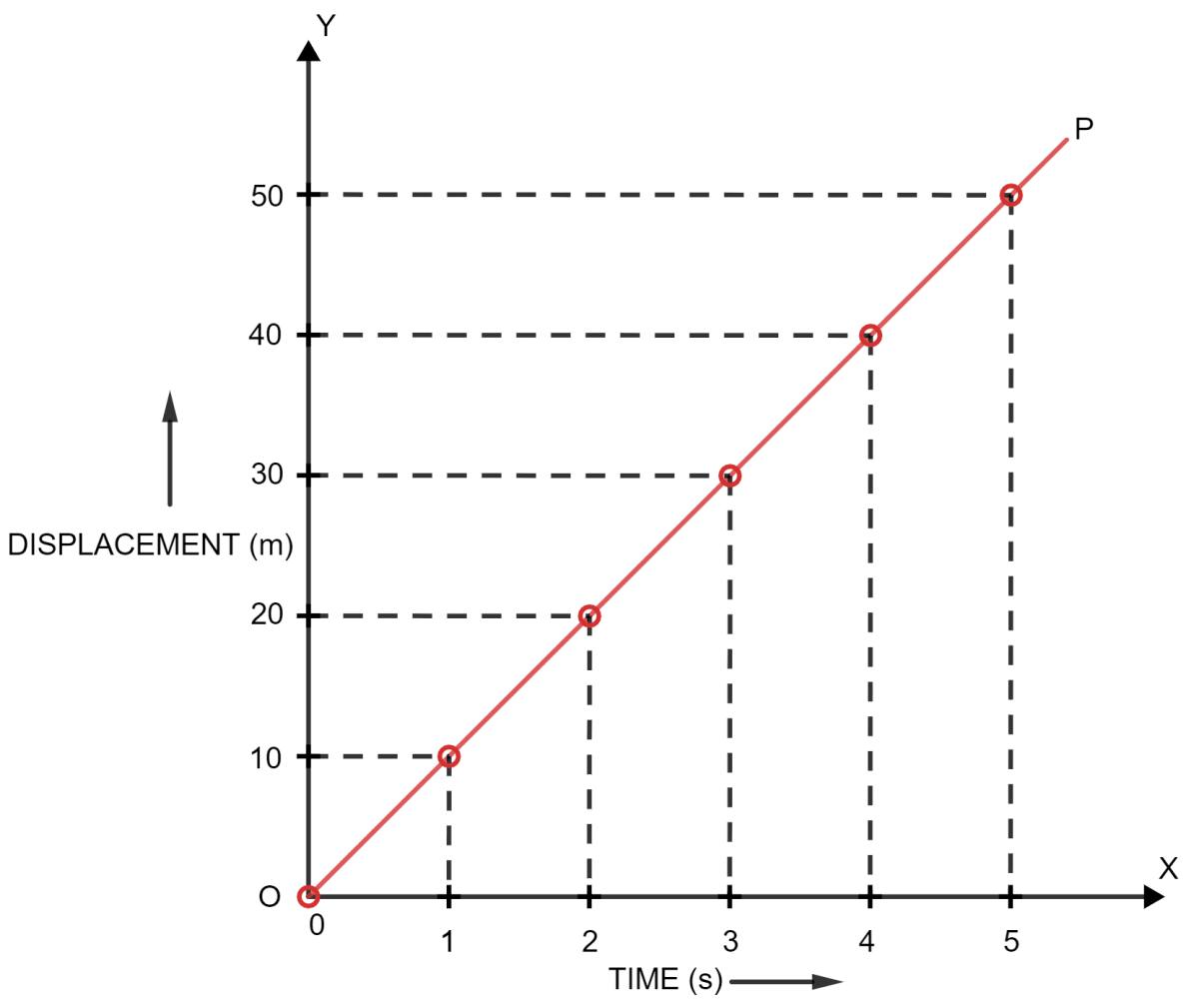#### Question 5

State how the velocity-time graph can be used to find —

(i) the acceleration of a body,

(ii) the distance travelled by the body in a given time, and

(iii) the displacement of the body in a given time.

(i) Acceleration of a body — As acceleration is equal to the ratio of change in velocity and time taken, therefore, the slope ( or gradient ) of the velocity-time graph gives the acceleration.

(ii) Distance travelled by the body in a given time — The total distance travelled by the body is obtained by the arithmetic sum of the positive displacement and negative displacement (without sign).

(iii) Displacement of the body — As the product of velocity and time gives the displacement, therefore, the area enclosed between the velocity-time sketch and X - axis (i.e., the time axis) gives the displacement of the body.

The total displacement is obtained by adding positive displacement and negative displacement numerically with proper sign.

#### Question 6

What can you say about the nature of motion of a body if its displacement-time graph is —

(a) a straight line parallel to time axis?

(b) a straight line inclined to the time axis with an acute angle?

(c) a straight line inclined to the time axis with an obtuse angle?

(d) a curve

The following can be said about the nature of motion of a body, if its displacement-time graph is as follows —

(a) A straight line parallel to time axis — shows that the body is stationary (or no motion).

(b) A straight line inclined to the time axis with an acute angle — shows the linear relationship between the displacement and time (i.e., the body travels equal distance in equal intervals of time in a certain direction). Hence, we can say the motion is away from the starting point with uniform velocity.

(c) A straight line inclined to the time axis with an obtuse angle — shows that there is motion towards the starting point with a uniform velocity.

(d) A curve — shows that the body moves with varying speed in a fixed direction. Hence, we can say that there is motion with variable velocity.

#### Question 7

The figure shows displacement-time graph of two vehicles A and B moving along a straight road. Which vehicle is moving faster? Give reason.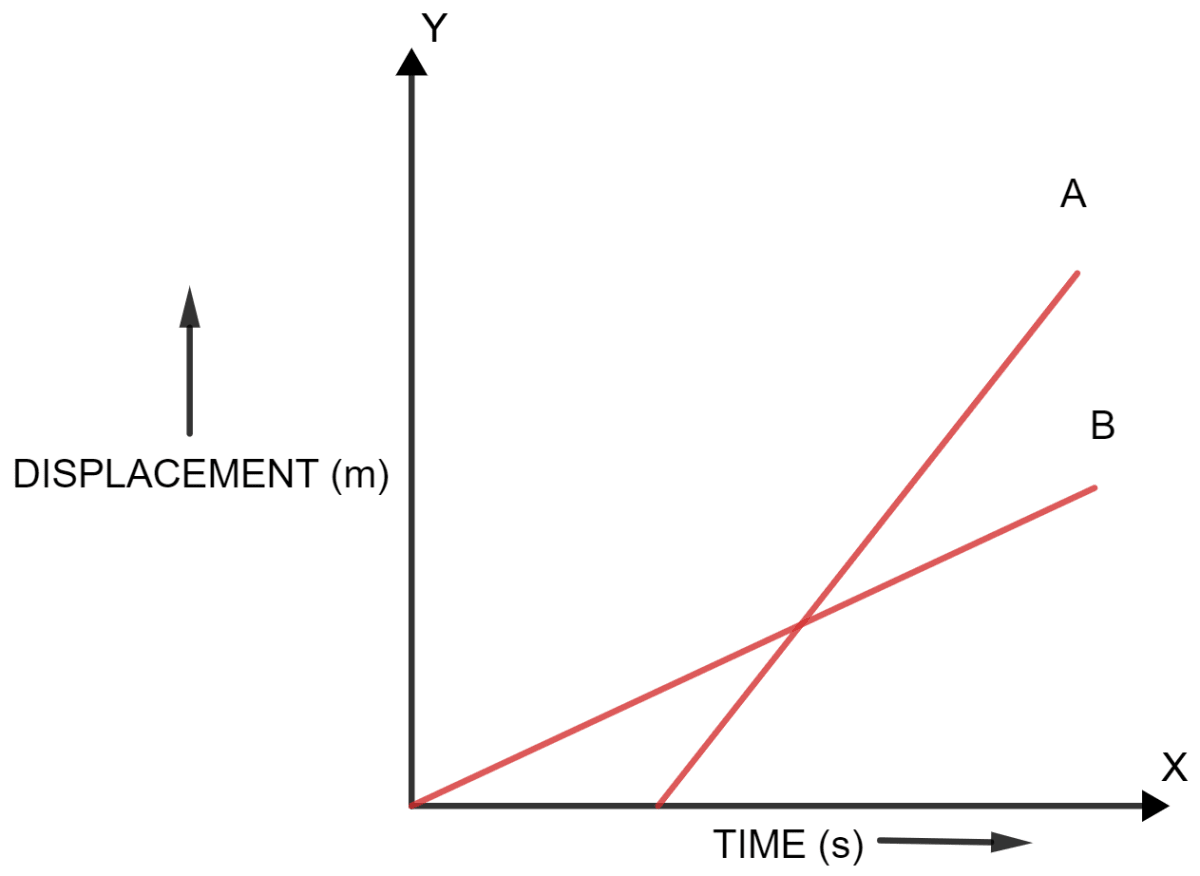We infer from the graph, that vehicle A is moving faster than vehicle B. It is so because, the slope of line A is more than that of line B.

#### Question 8

State the type of motion represented by the following sketches in the figure.

Give example of each type of motion.

(a)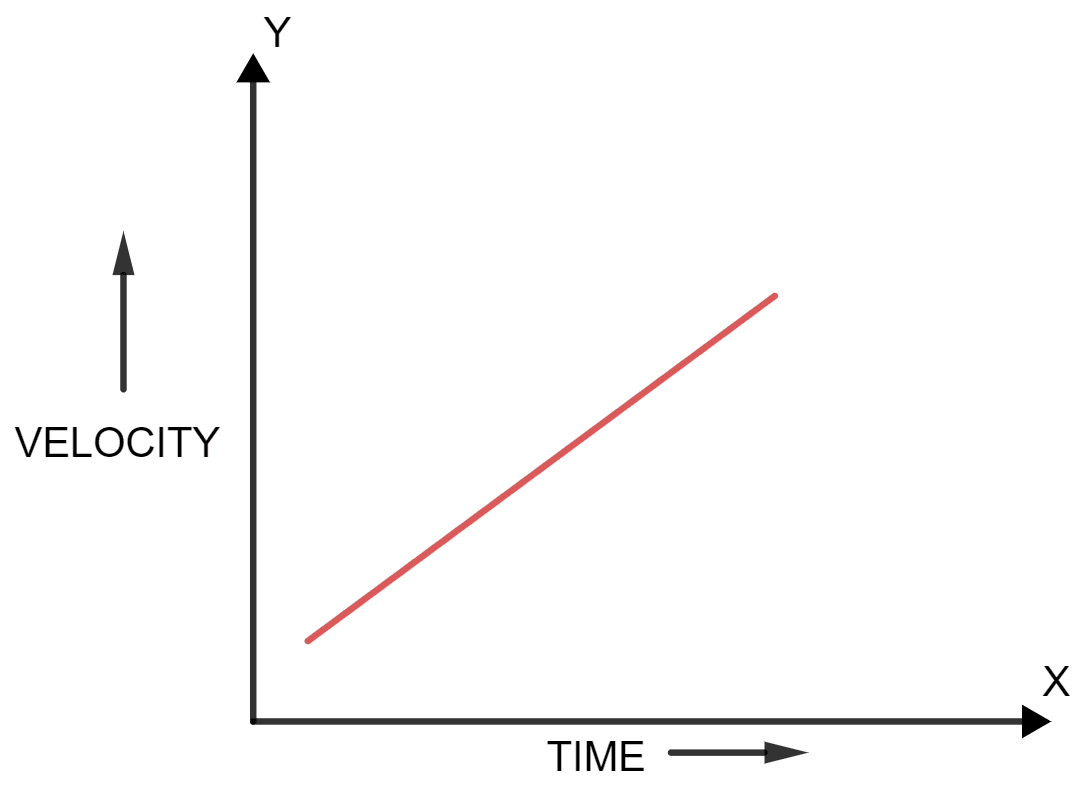(b)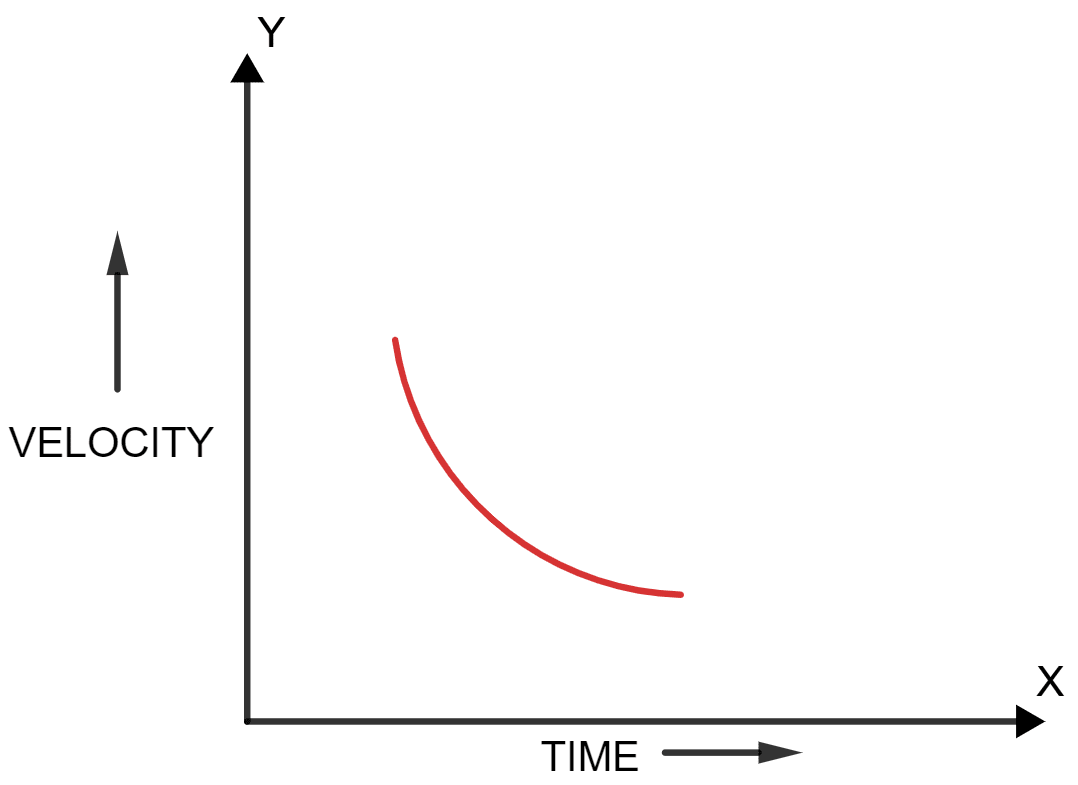(a) The graph shows uniformly accelerated motion.

Example — Uniform acceleration is depicted by the motion of a body released downwards.

(b) The graph shows the motion with a variable retardation.

Example — Motion with a variable retardation is shown by a car approaching its destination.

#### Question 9

Draw a velocity-time graph for a body moving with an initial velocity u and uniform acceleration a. Use this graph to find the distance travelled by the body in time t.

Below is the velocity-time graph for a body moving with an initial velocity u and uniform acceleration a: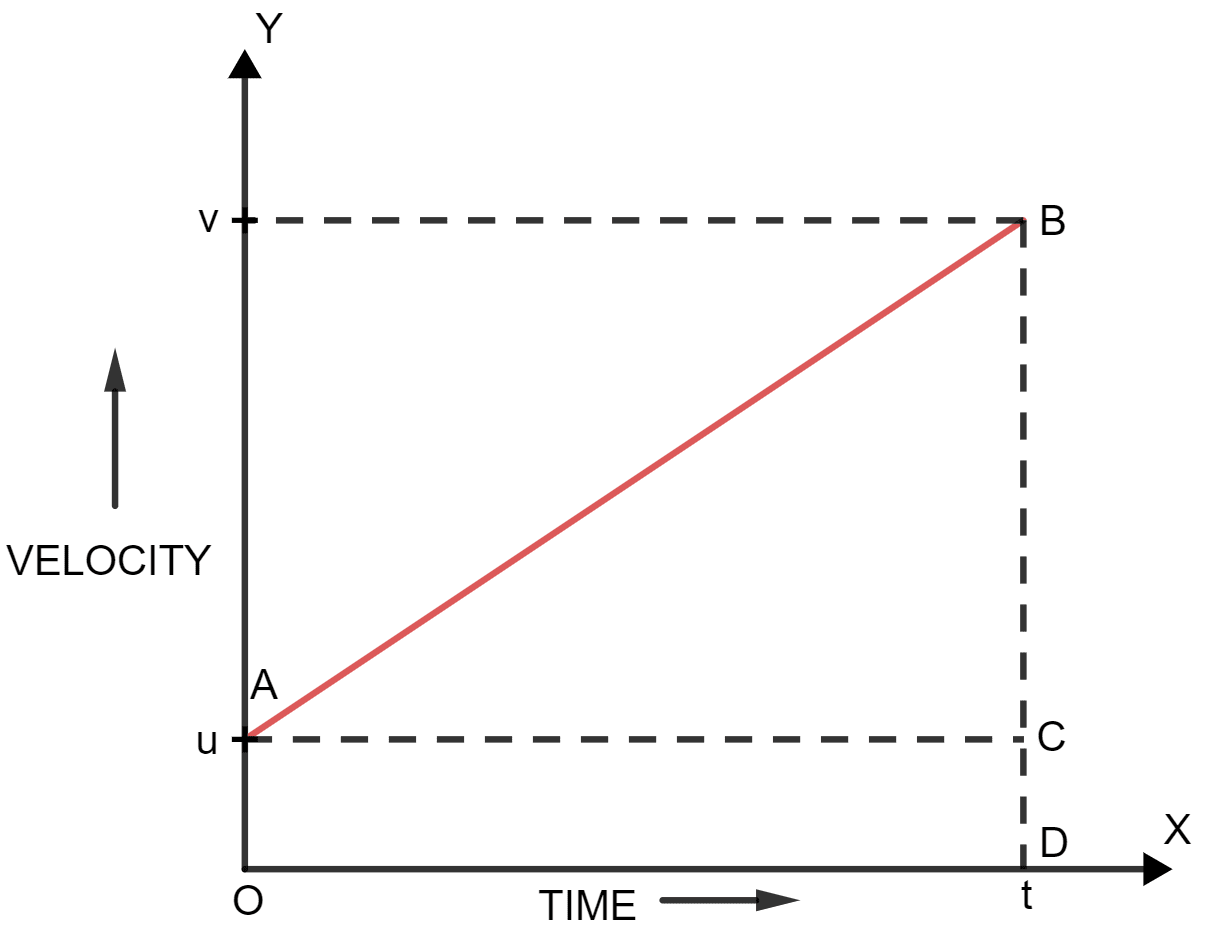Let v be the velocity at time t for this body having initial velocity u and uniform acceleration a as shown in the graph.

Distance travelled by the body in time t = Area under the velocity-time curve.

Hence,

Distance travelled = area of trapezium OABD

= $\dfrac{1}{2}$ x sum of parallel sides x height

= $\dfrac{1}{2}$ x (OA + DB) x OD

= $\dfrac{1}{2}$ x (u + v) x t

= $\dfrac{(\text{u} + \text{v})\text{t}}{2}$

#### Question 10

What does the slope of velocity-time graph represent?

As the ratio of change in velocity and time taken is equal to the acceleration. Therefore,the slope of the velocity-time graph represents the acceleration.

#### Question 11

Figure shows the velocity-time graph for two cars A and B moving in same direction. Which car has the greater acceleration? Give reason to your answer.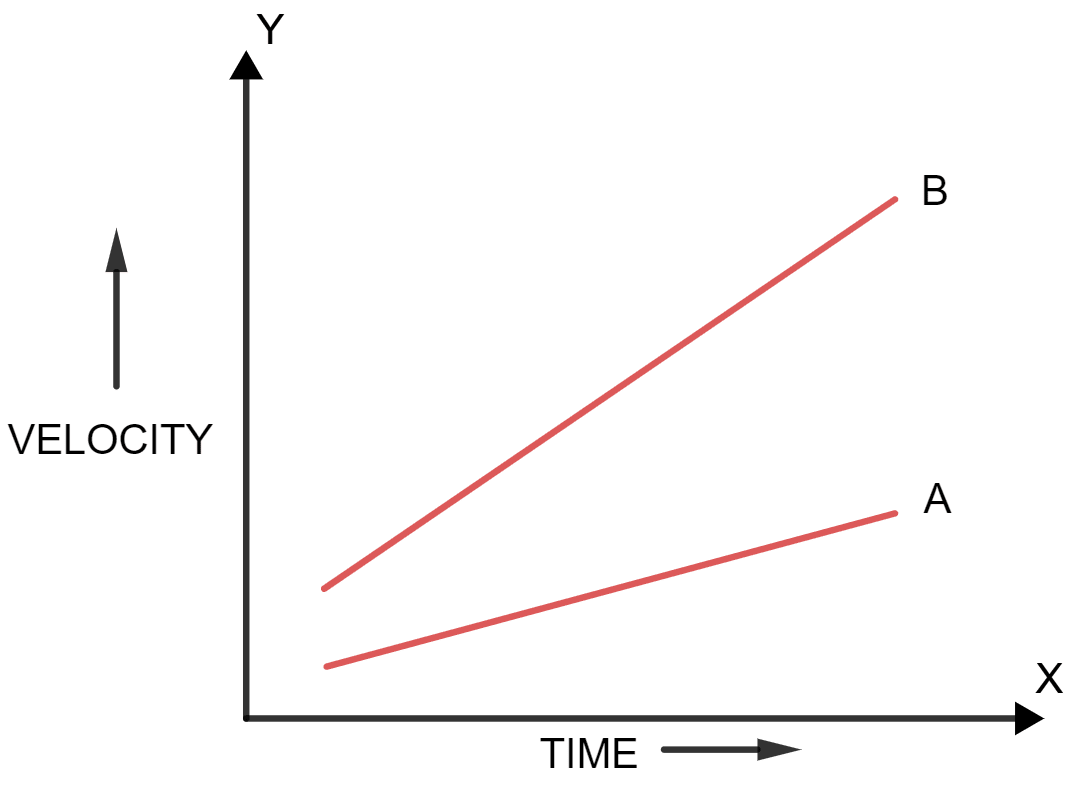We can infer from the graph, that the car B has greater acceleration than car A as the slope of straight line for car B is more than that of car A.

#### Question 12

Draw the velocity-time graph for a body moving with —

(a) uniform velocity,

(b) uniform acceleration.

(a) Below is the velocity-time graph for a body moving with uniform velocity: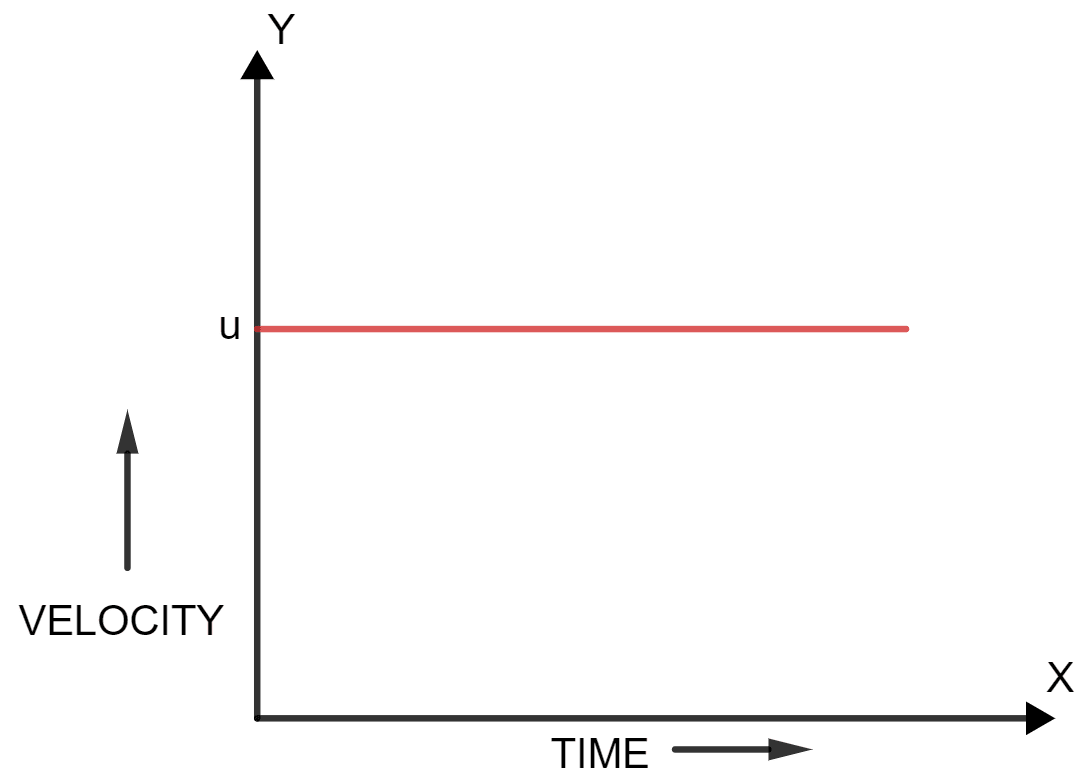(b) Below is the velocity-time graph for a body moving with uniform acceleration:#### Question 13

The velocity-time graph for a uniformly retarded body is a straight line inclined to the time axis with an obtuse angle. How is retardation calculated from the velocity-time graph?

Retardation is calculated from the velocity-time graph, by finding the negative slope.

#### Question 14

Figure shows the displacement-time graph for four bodies A, B, C and D. In each case state what information do you get about the acceleration (zero, positive or negative).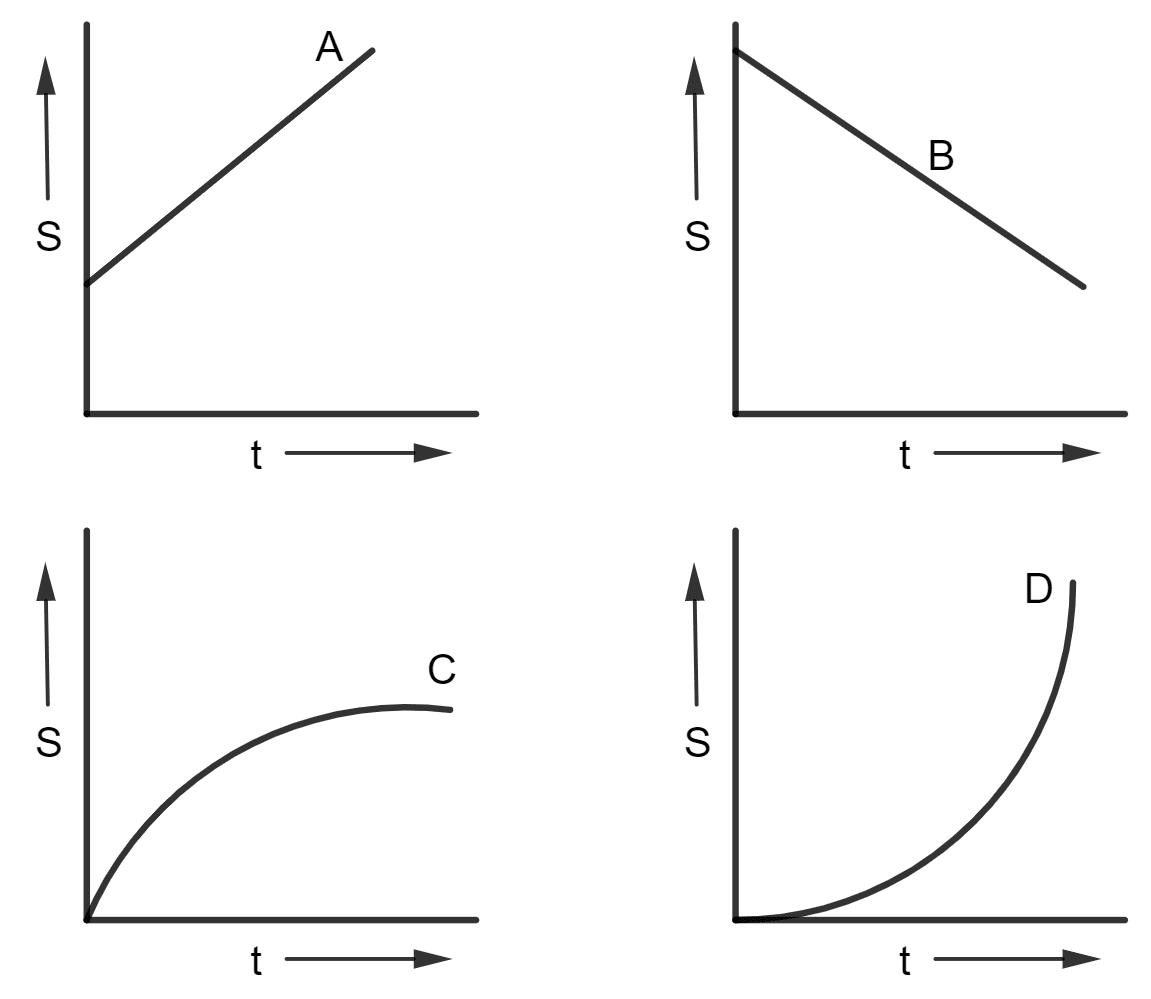The following information is obtained from the given figures —

• A → Zero acceleration since slope (i.e., velocity) is constant.
• B → Zero acceleration since slope (i.e., velocity) is constant.
• C → Negative acceleration (or retardation) since slope is decreasing with time.
• D → positive acceleration since slope is increasing with time.

#### Question 15

Draw a graph for acceleration against time for a uniformly accelerated motion. How can it be used to find the change in speed in a certain interval of time?

Below is the graph for acceleration against time for a uniformly accelerated motion: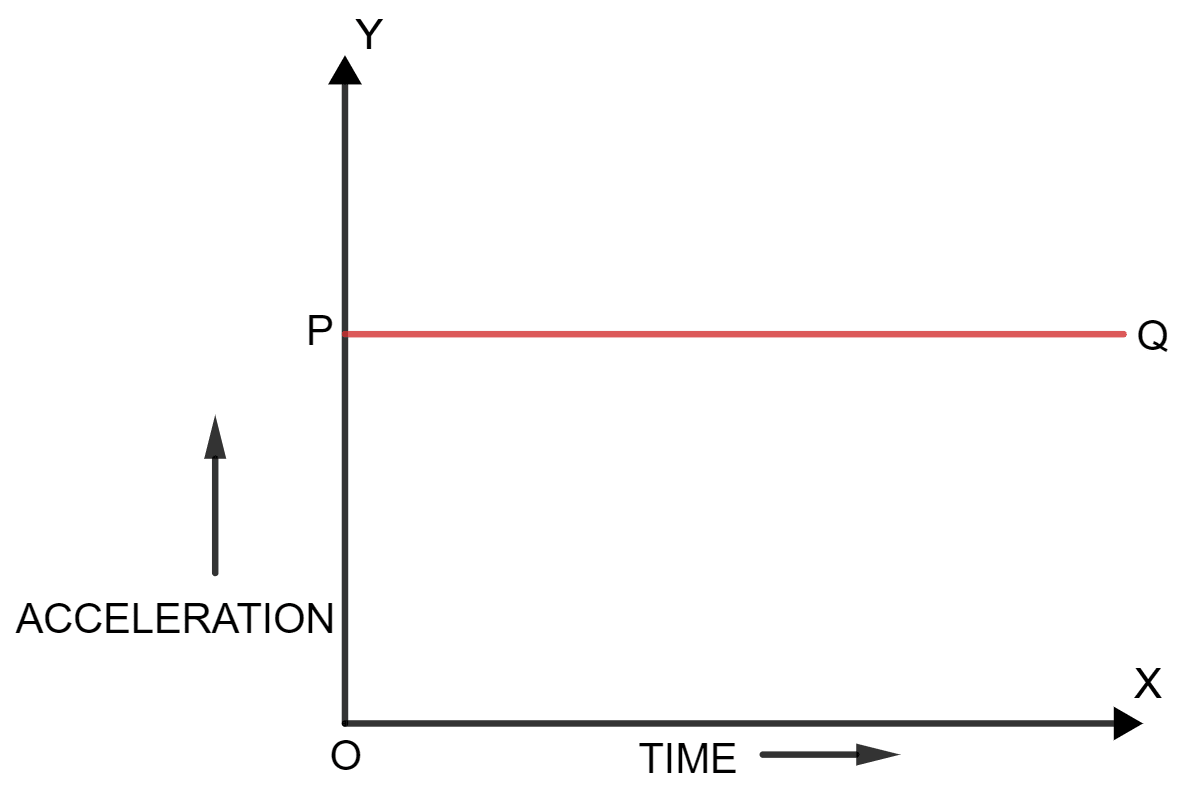For linear motion,

Acceleration x time = change in speed

Therefore, we can find the change in speed from the area enclosed between the acceleration-time sketch and the time axis.

#### Question 16

Draw a velocity-time graph for the free fall of a body under gravity, starting from rest.

Take g = 10 m s-2.

Below is the velocity-time graph for the free fall of a body under gravity, starting from rest: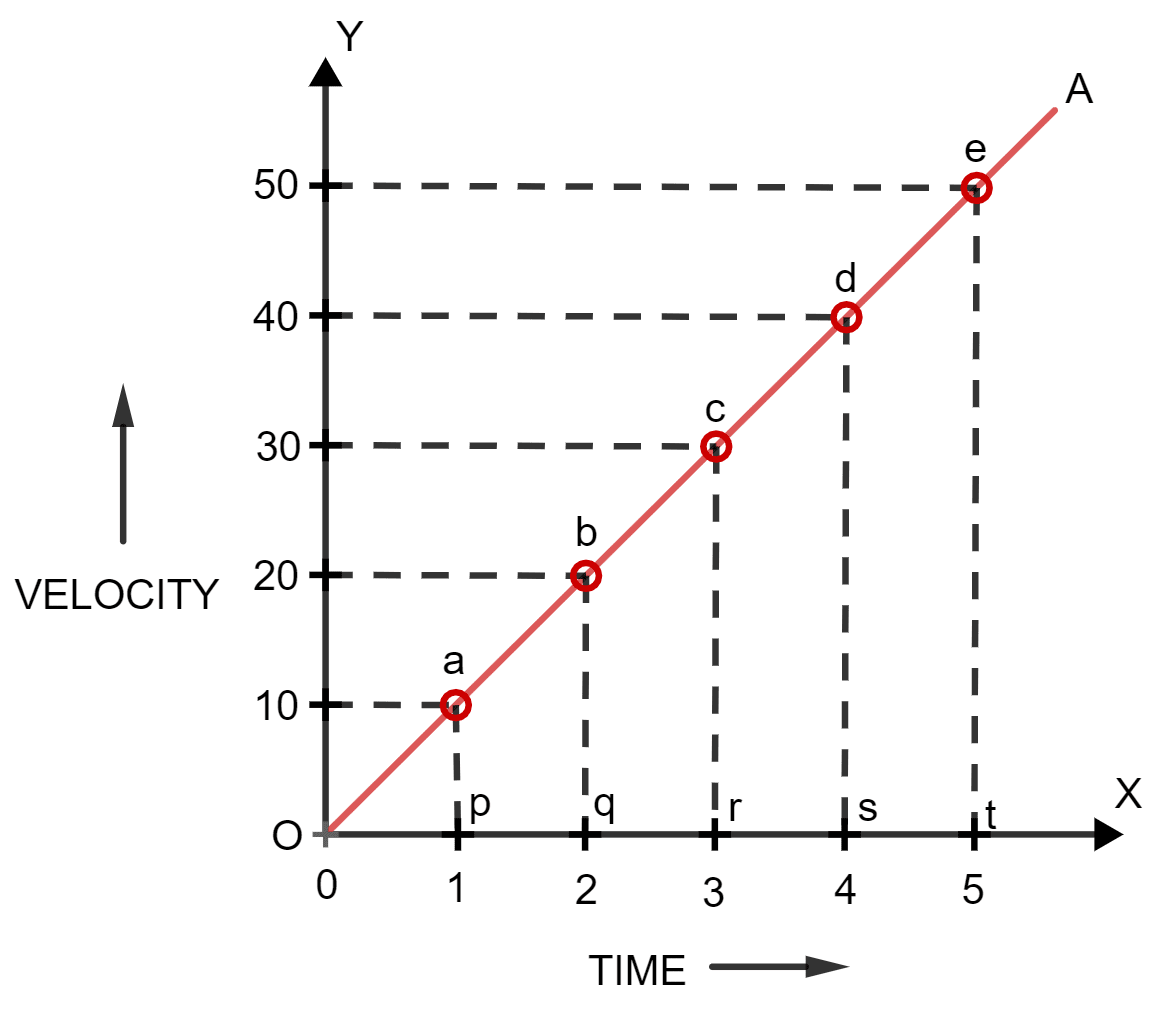#### Question 17

How is the distance related with time for the motion under uniform acceleration such as the motion of a freely falling body ?

For a freely falling body (i.e., motion under uniform acceleration ), the displacement is directly proportional to the square of time.

S ∝ t2

#### Question 18

A body falls freely from a certain height. Show graphically the relation between the distance fallen and square of time. How will you determine g from this graph?

Below is the graph of distance fallen against square of time for a freely falling body: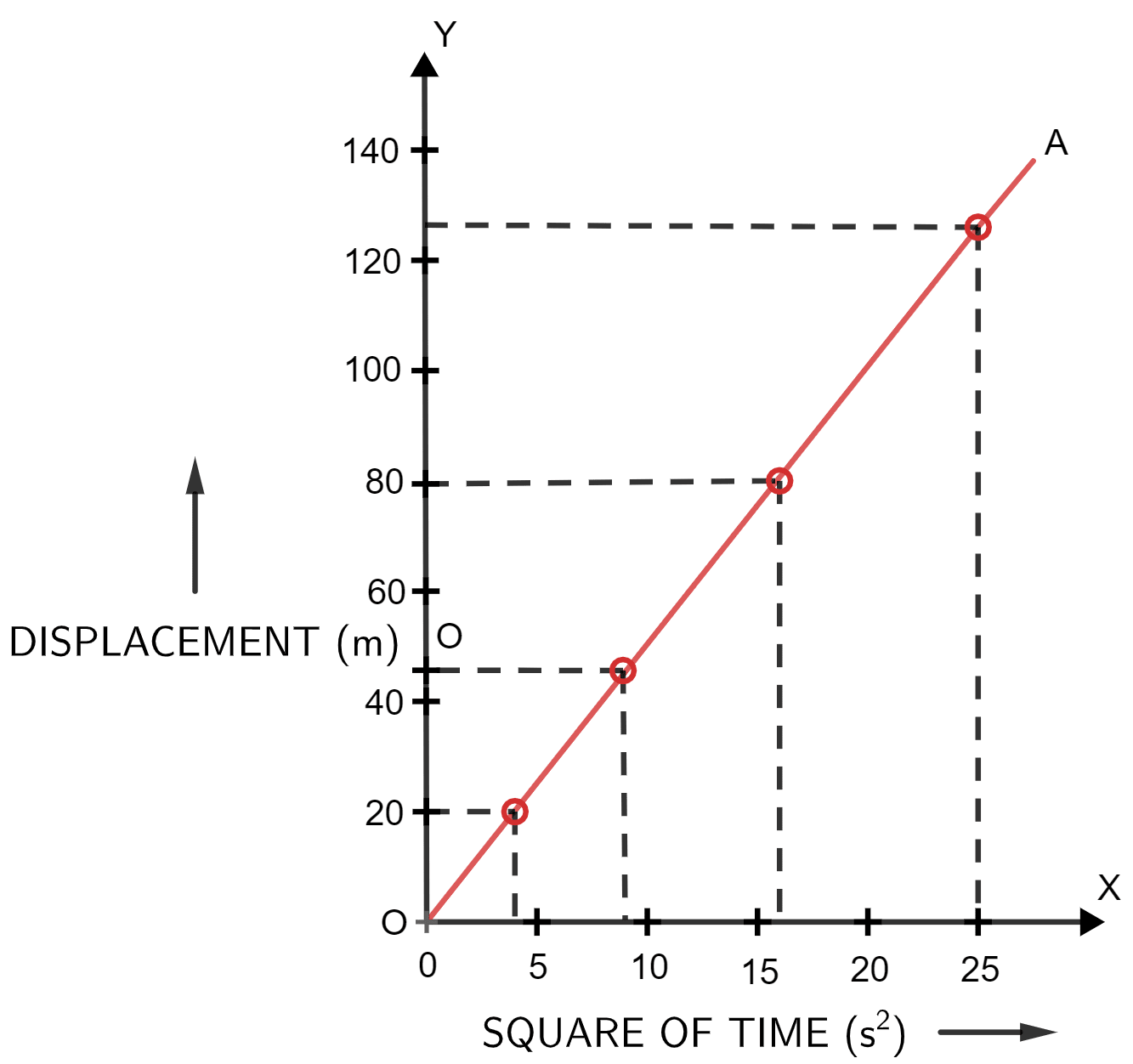The slope is half the acceleration due to gravity.

Thus, the value of acceleration due to gravity (g) can be obtained by doubling the slope of the S - t2 graph for a freely falling body.

## Multiple Choice Type

#### Question 1

The velocity-time graph of a body in motion is a straight line inclined to the time axis. The correct statement is —

1. Velocity is uniform
2. Acceleration is uniform ✓
3. Both velocity and acceleration are uniform
4. Neither velocity nor acceleration is uniform.

When the velocity-time graph of a body in motion is a straight line inclined to the time axis then there are equal changes in velocity in equal intervals of time and hence, the movement is with uniform acceleration.

#### Question 2

For a uniformly retarded motion, the velocity-time graph is —

1. A curve
2. A straight line parallel to the time axis
3. A straight line perpendicular to the time axis.
4. A straight line inclined to the time axis. ✓

If the velocity of a body decreases at a constant rate (i.e., motion is uniformly retarded), the velocity-time graph is a straight line inclined to the time axis and it is a negative slope.

#### Question 3

For uniform motion —

1. The distance-time graph is a straight line parallel to the time axis
2. The speed-time graph is a straight line inclined to the time axis
3. The speed-time graph is a straight line parallel to the time axis ✓
4. The acceleration-time graph is a straight line parallel to the time axis.

The speed-time graph is a straight line parallel to the time axis, for a uniform motion as the slope is zero and hence the acceleration is zero and motion is uniform.

## Numericals

#### Question 1

Figure (a) shows the displacement-time graph for the motion of a body. Use it to calculate the velocity of body at t = 1 s, 2 s and 3 s, then draw the velocity-time graph for it in Figure (b).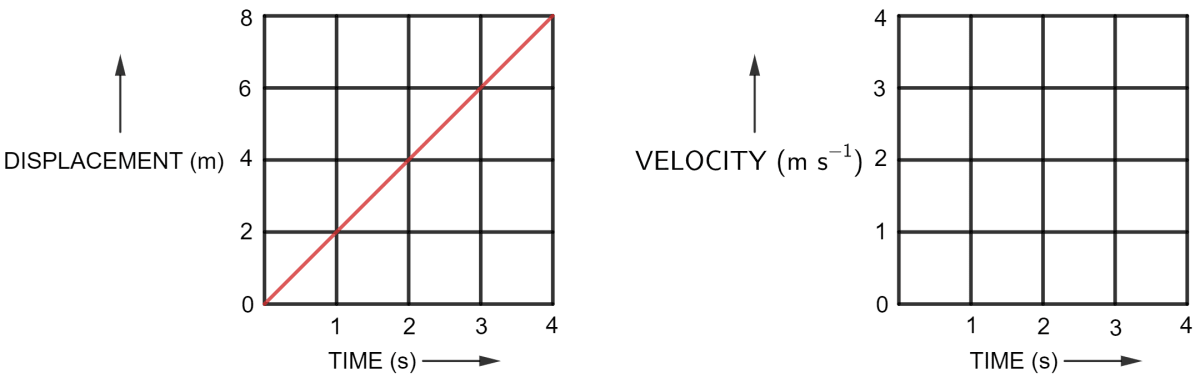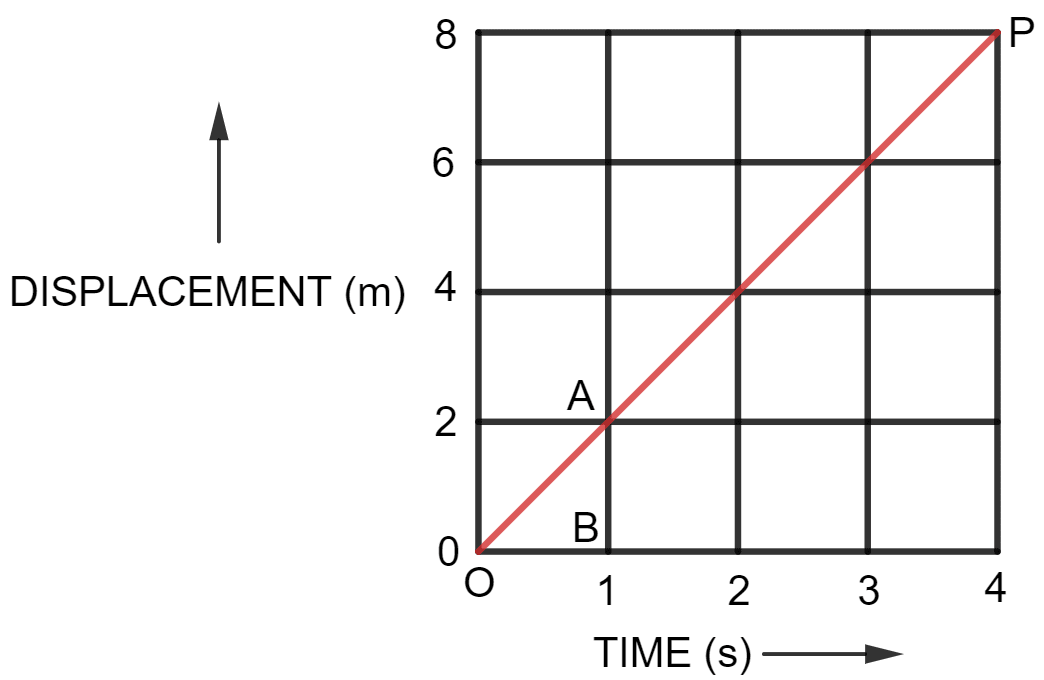We observe from the given displacement time graph above, that the slope is a straight line inclined with time axis, so the body is moving with uniform velocity. Hence, the velocity will be same at t = 1 s, 2 s and 3 s.

$\text{Velocity} = \text{Slope of straight line OP} \\[0.5em] = \dfrac{\text{AB}}{\text{OB}} \\[0.5em] = \dfrac{(2 - 0) \text{ m}}{(1 - 0) \text{ s}} \\[0.5em] = \dfrac{2 \text{ m}}{1 \text{ s}} \\[0.5em] = 2 \text{ m s}^{-1}$

Hence, velocity at t = 1 s, 2 s and 3 s is equal to 2 m s-1

Velocity-time graph for the motion of a body is given below: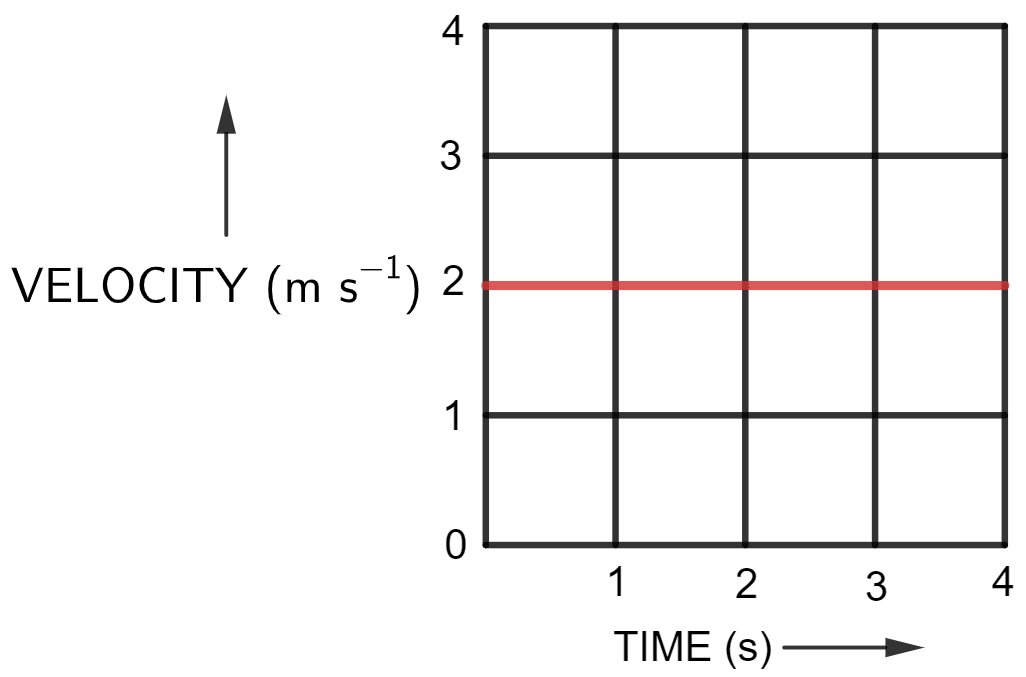#### Question 2

Following table gives the displacement of a car at different instants of time.

Time (s)01234
Displacement (m)05101520

(a) Draw the displacement-time sketch and find the average velocity of car.

(b) What will be the displacement of car at (i) 2.5 s and (ii) 4.5 s ?

(a) The displacement-time sketch for the car is shown below: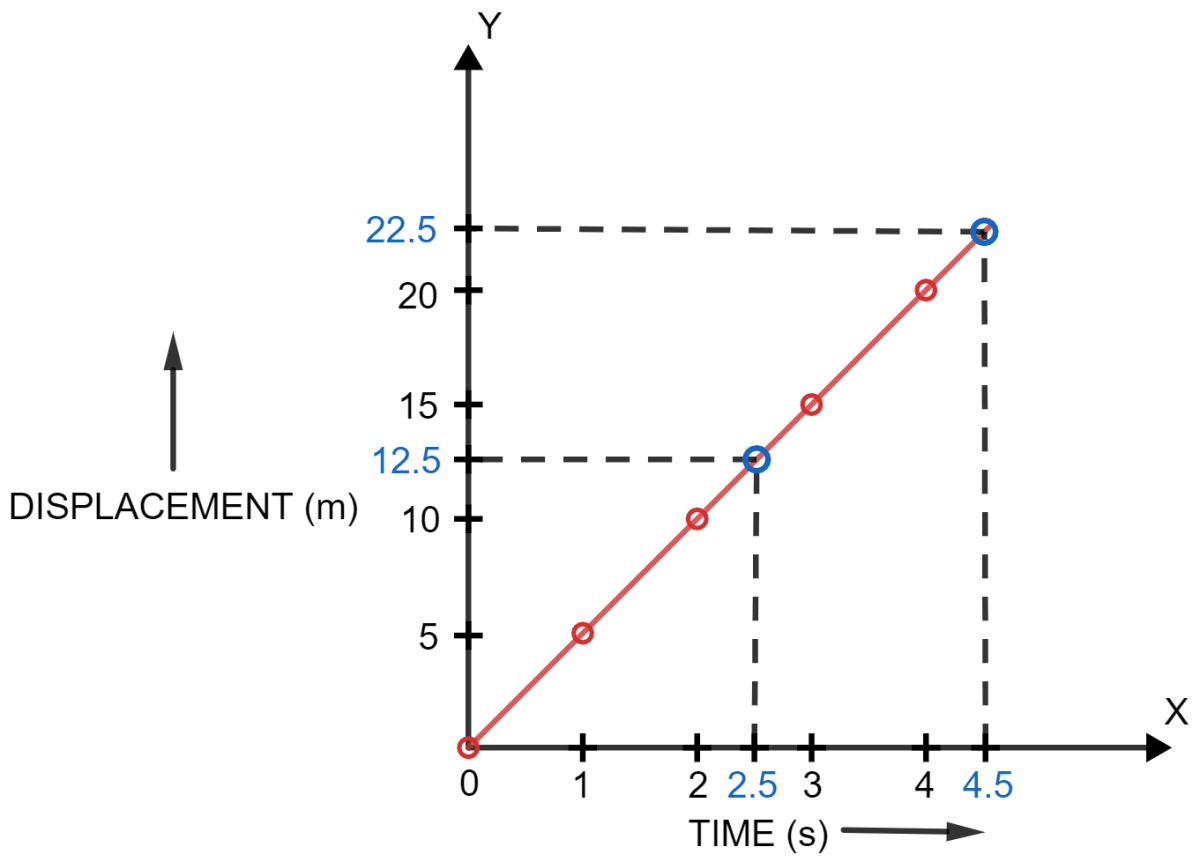As we know,

$\text {Avg. velocity of car} = \dfrac {\text {Total distance}}{\text {Total time}} \\[1em] \text {Avg. velocity of car} = \dfrac {20 - 0 \text { m}}{4 - 0\text { s}} \\[1em] \text {Avg. velocity of car} = \dfrac {20 \text { m}}{4\text { s}} \\[1em] \Rightarrow \text {Avg. velocity of car} = 5\text { ms} ^ {-1}$

Hence, average velocity of the car is 5 ms-1.

(b) When we observe the graph, we can find the displacement of the car at various points,

At t = 2.5 s, displacement = 12.5 m and

At t = 4.5 s, displacement = 22.5 m

#### Question 3

A body is moving in a straight line and its displacement at various instants of time is given in the following table —

Time (s)01234567
Displacement (m)26121212182224

Plot displacement-time graph and calculate —

(i) Total distance travelled in interval 1 s to 5 s,

(ii) Average velocity in time interval 1 s to 5 s.

The displacement-time graph is given below: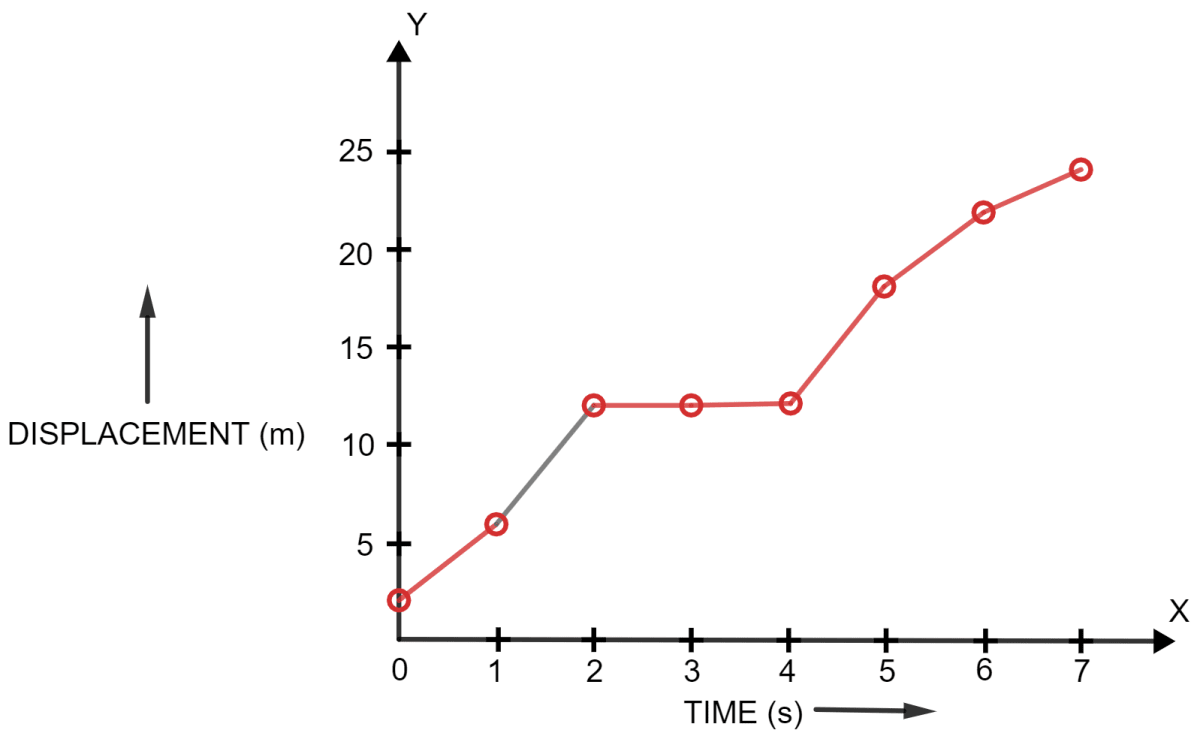(i) When we observe the graph, we find —

Total distance travelled in interval 1 s to 5 s = 18 m - 6 m = 12 m

Hence, total distance travelled in interval 1 s to 5 s = 12 m

(ii) As we know,

$\text {Avg. velocity} = \dfrac {\text {Total distance}}{\text {Total time}} \\[1em] \text {Avg. velocity} = \dfrac {18 - 6 \text {m}}{5 - 1\text {s}} \\[1em] \text {Avg. velocity} = \dfrac {12 \text {m}}{4\text {s}} \\[1em] \Rightarrow \text {Avg. velocity} = 3\text {ms} ^ {-1}$

Hence, average velocity of the car in interval 1 s to 5 s is 3 ms-1.

#### Question 4

Figure shows the displacement of a body at different times.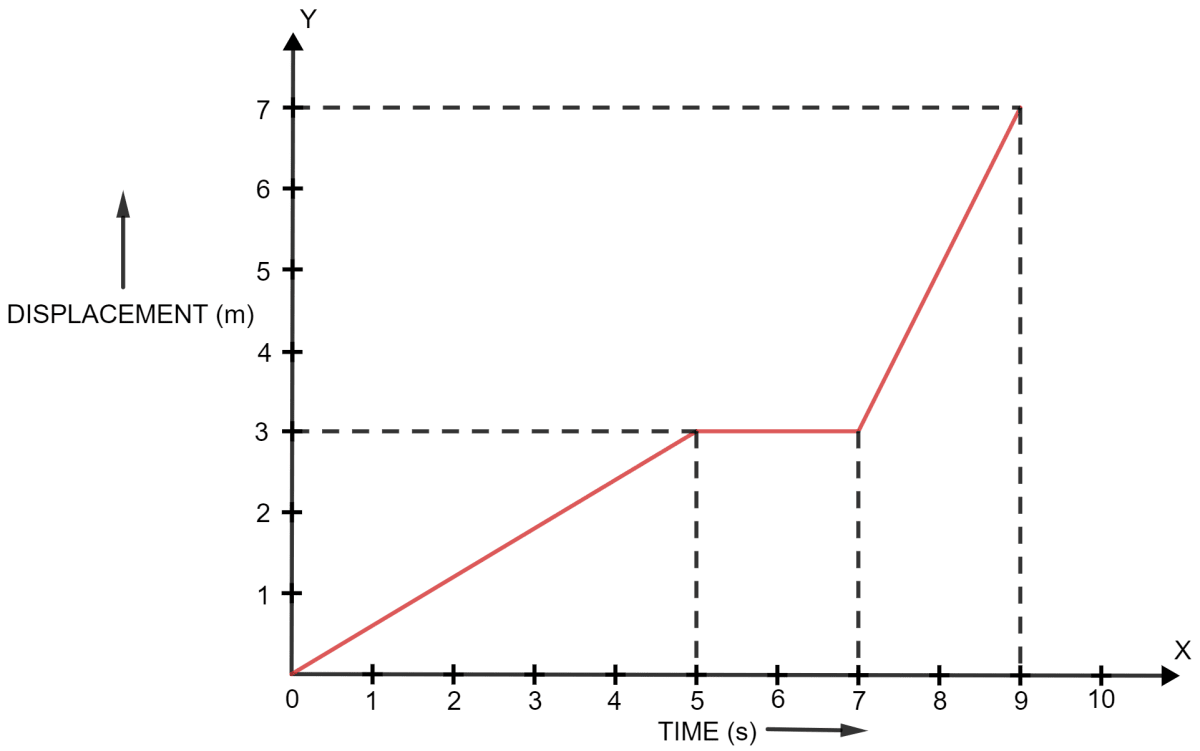(a) Calculate the velocity of the body as it moves for time interval

(i) 0 to 5 s,

(ii) 5 s to 7 s and

(iii) 7 s to 9 s.

(b) Calculate the average velocity during the time interval 5 s to 9 s.

[ Hint — From 5 s to 9 s, displacement = 7 m - 3 m = 4 m ]

Below is the displacement-time graph of the body with the different points marked: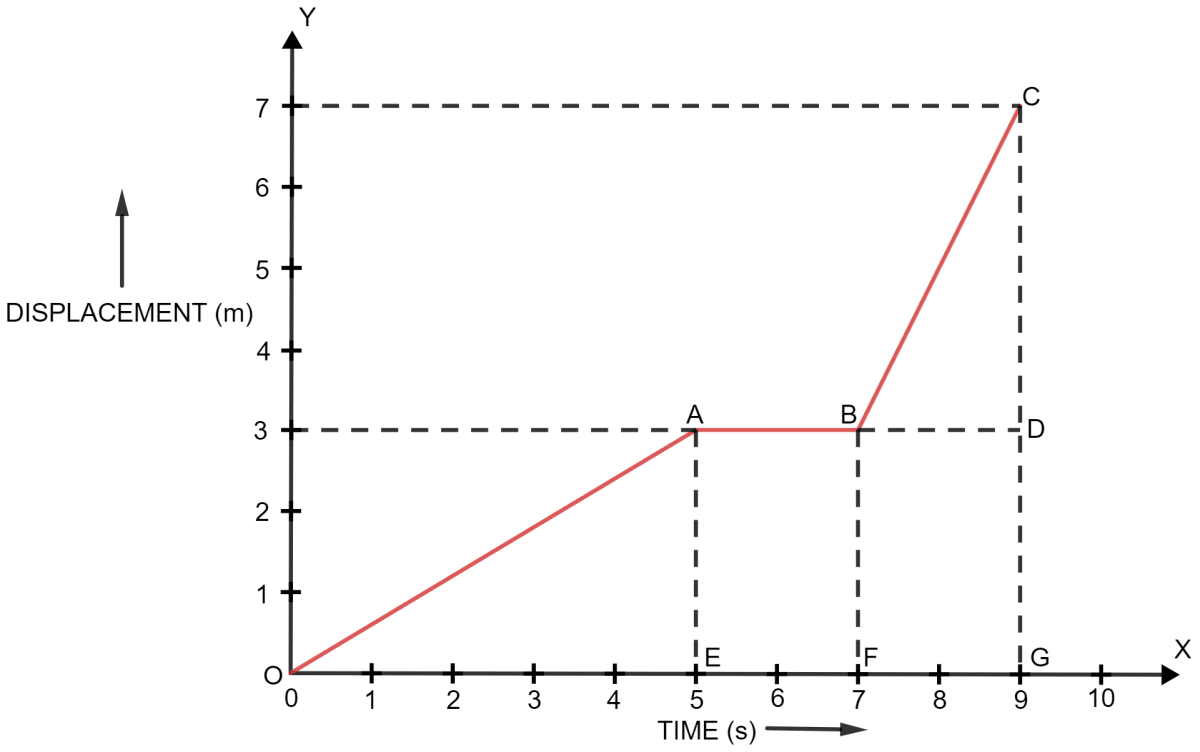(a) As we know,

(i) At t = 0 to 5 s

Velocity = Slope of straight line OA

$\text {Velocity} = \dfrac {\text {AE}}{\text {OE}} = \dfrac {3 - 0 \text {m}}{5 - 0 \text {s}} \\[0.5em] \Rightarrow \text {Velocity} = \dfrac {3 \text {m}}{5 \text {s}} \\[0.5em] \Rightarrow \text {Velocity} = 0.6 {\text {ms}}^{-1} \\[0.5em]$

Hence, velocity at t = 0 to 5 s = 0.6 m s-1

(ii) At t = 5 to 7 s

In this part we observe that there is no change in Y axis, (i.e. displacement is zero so the body is stationary).

Hence, velocity at t = 5 to 7 s = 0 m s-1

(iii) At t = 7 s to 9 s

Velocity = Slope of straight line BC

$\text {Velocity} = \dfrac {\text {CD}}{\text {BD}} = \dfrac {7 - 3 \text {m}}{9 - 7 \text {s}} \\[0.5em] \Rightarrow \text {Velocity} = \dfrac {4 \text {m}}{2 \text {s}} \\[0.5em] \Rightarrow \text {Velocity} = 2 {\text {ms}}^{-1} \\[0.5em]$

Hence, velocity at t = 7 s to 9 s = 2 m s-1

(b) As we know,

$\text {Avg. velocity} = \dfrac {\text {Total distance}}{\text {Total time}} \\[0.5em]$

Substituting the values from the graph we get,

$\text {Avg. velocity} = \dfrac {7 - 3 \text {m}}{9 - 5\text {s}} \\[0.5em] \text {Avg. velocity} = \dfrac {4 \text {m}}{4\text {s}} \\[0.5em] \Rightarrow \text {Avg. velocity} = 1\text {ms} ^ {-1} \\[0.5em]$

Hence, average velocity of the car is 1 ms-1.

#### Question 5

From the displacement-time graph of a cyclist, given in figure, find —

(i) The average velocity in the first 4 s,

(ii) The displacement from the initial position at the end of 10 s

(iii) The time after which he reaches the starting point.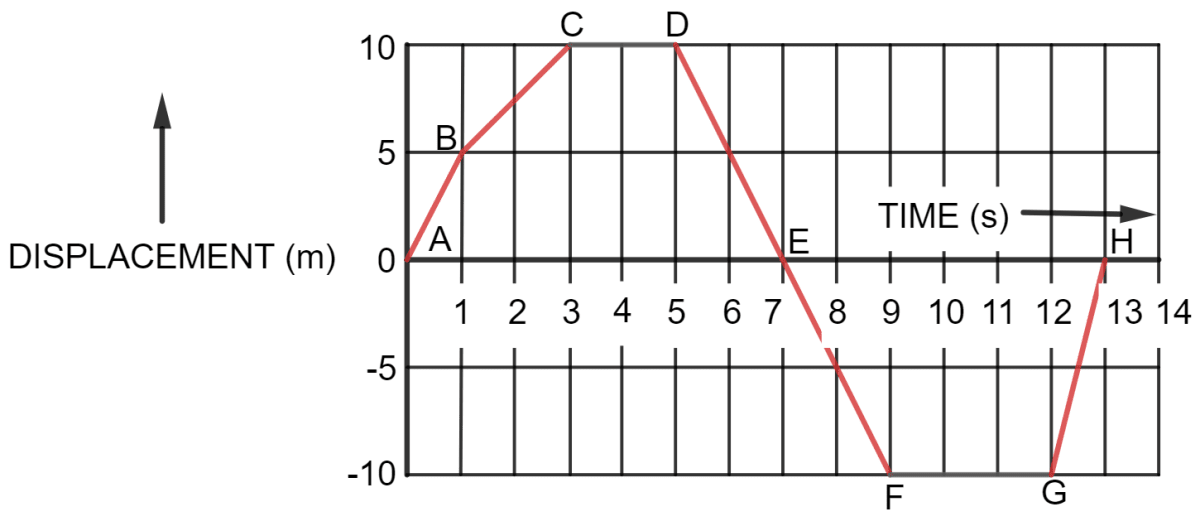(i) As we know,

$\text {Avg. velocity} = \dfrac {\text {Total distance}}{\text {Total time}}$

Substituting the values from the graph, for the first 4 s, we get,

$\text {Avg. velocity} = \dfrac {10 \text {m}}{4 \text {s}} \\[0.5em] \Rightarrow \text {Avg. velocity} = 2.5\text {ms} ^ {-1} \\[0.5em]$

Hence, average velocity of the car is 2.5 ms-1.

(ii) From the graph, we get,

Displacement = final position - initial position

Substituting the values from the graph, for the first 10 s, we get,

Displacement = -10 - 0 = -10 m

Hence, displacement from the initial position at the end of 10 s = - 10 m

(iii) The cyclist would reach the start point two times, one at the 7 s and the other at 13 s.

#### Question 6

Figure ahead represents the displacement-time sketch of motion of two cars A and B.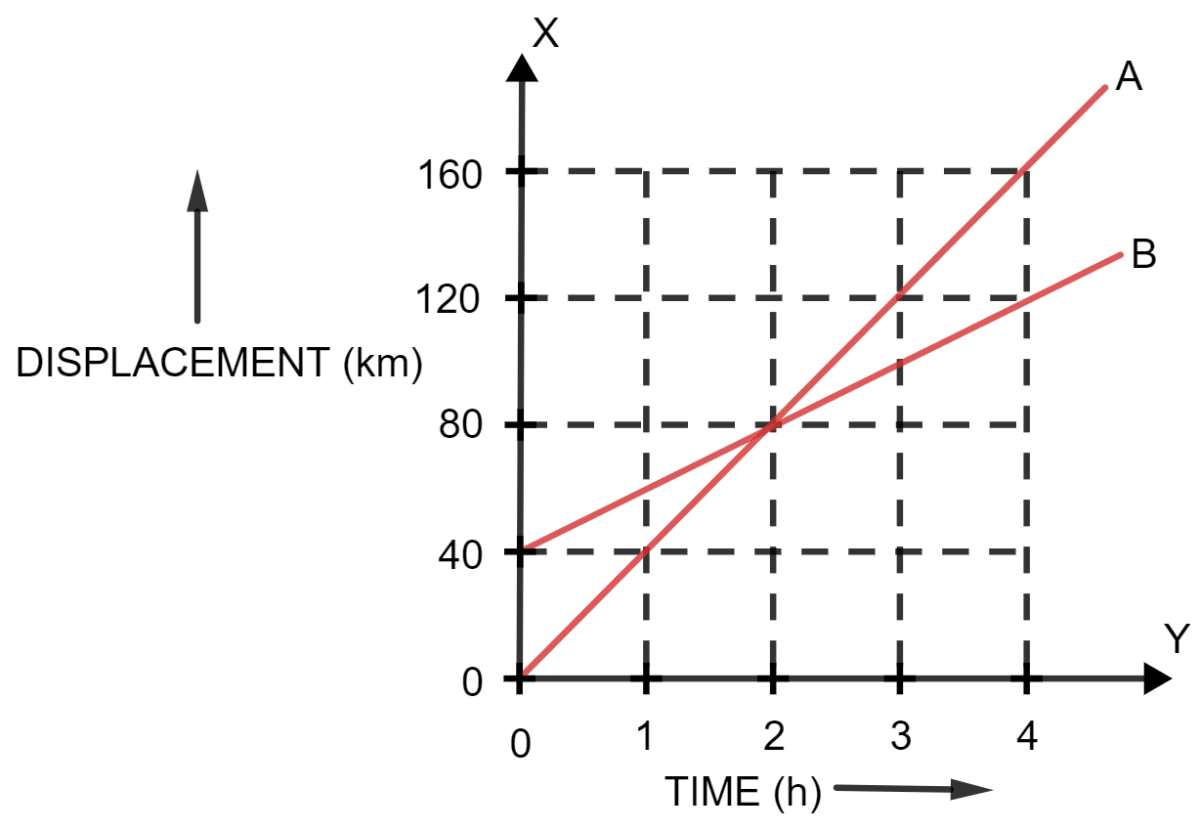Find —

(i) The distance by which the car B was initially ahead of car A.

(ii) The velocities of car A and car B

(iii) The time in which car A catches car B

(iv) The distance from start when the car A will catch the car B

(i) When we observe the graph, we find that the car B was ahead of car A by 40 km.

(ii) As we know,

$\text{Velocity} = \dfrac{\text{displacement}}{\text{time}}$

Cars A and B have uniform velocities as displacement-time graph for both are straight lines.

At t = 4 h

Car A

$\text {Velocity} = \dfrac {160 - 0 \text {km}}{4 - 0 \text {h}} \\[0.5em] \Rightarrow \text {Velocity} = \dfrac {160 \text {km}}{4 \text {h}} \\[0.5em] \Rightarrow \text {Velocity} = 40 {\text {kmh}}^{-1} \\[0.5em]$

Hence, velocity of car A = 40 km h-1

Car B

$\text {Velocity} = \dfrac {120 - 40 \text {km}}{4 - 0 \text {h}} \\[0.5em] \Rightarrow \text {Velocity} = \dfrac {80 \text {km}}{4 \text {h}} \\[0.5em] \Rightarrow \text {Velocity} = 20 {\text {kmh}}^{-1} \\[0.5em]$

Hence, velocity of car B = 20 km h-1

When we observe the graph, we find that both cars A and B intersect at a point K.

Hence,

(iii) the car A catches car B at time (t) = 2 h.

(iv) the distance from start when the car A will catch the car B = 80 km.

#### Question 7

A body at rest is made to fall from the top of a tower. Its displacement at different instants is given in the following table —

Time (in s)0.10.20.30.40.50.6
Displacement (in m)0.050.200.450.801.251.80

Draw a displacement-time graph and state whether the motion is uniform or non-uniform?

Below is the displacement-time graph of the body made to fall from the top of a tower: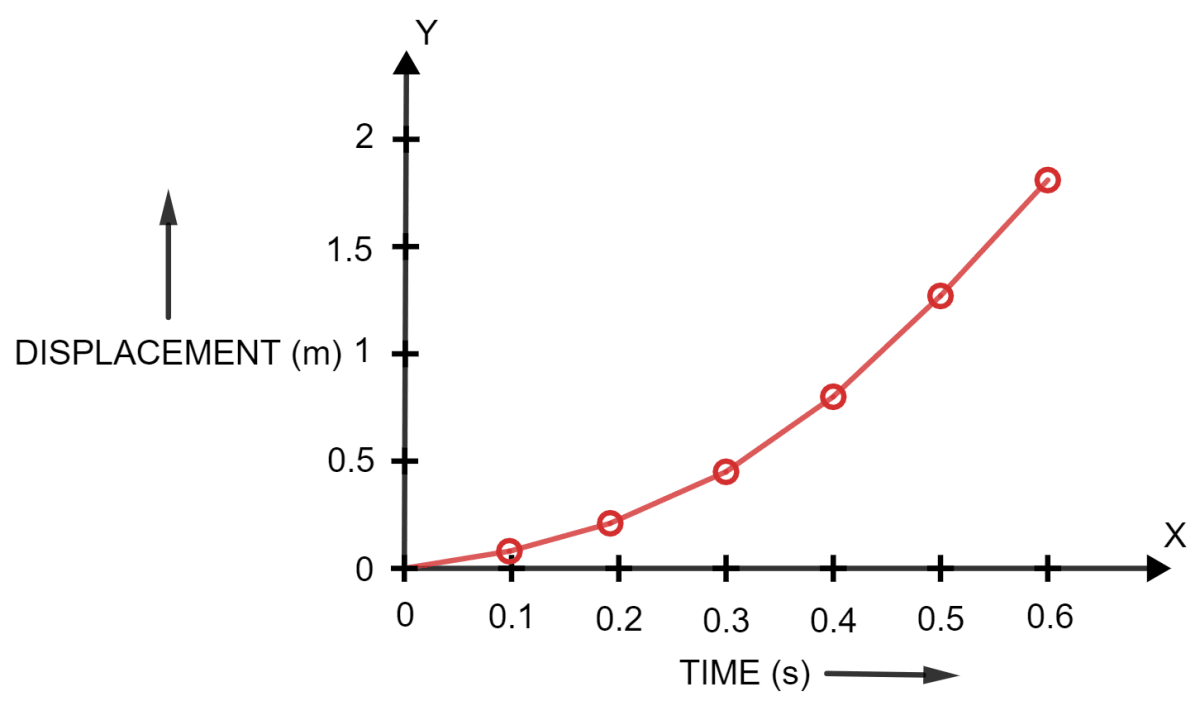When we observe the graph, we find, that the displacement-time graph is a curve.

Hence, we can say that the motion is non-uniform.

#### Question 8

Figure shows the velocity-time graph for the motion of a body. Use it to find the displacement of the body at t = 1 s, 2 s, 3 s and 4 s, then draw the displacement-time graph for it on figure (b).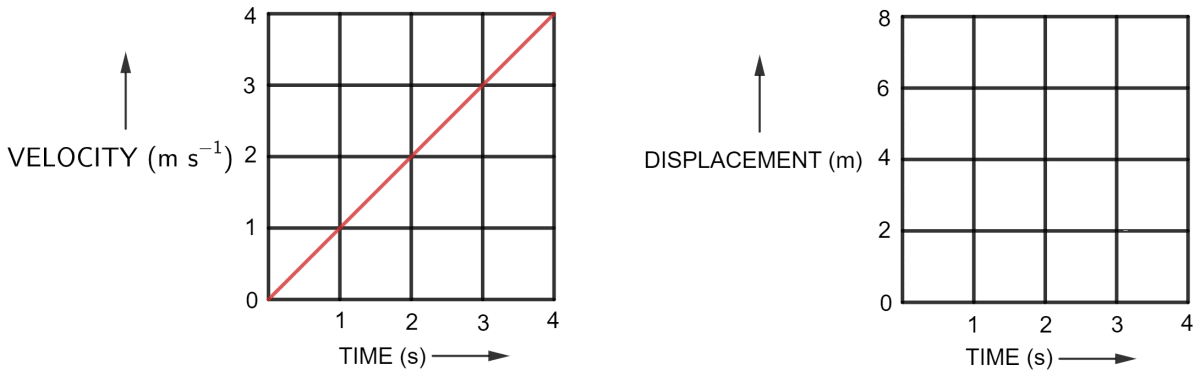As we know, the displacement of body at any instant can be obtained by finding the area enclosed by the straight line with the time axis up to that instant.

Let displacements at t = 1 s, 2 s, 3 s, 4 s be S1, S2, S3, S4 respectively.

At t = 1 s,

S1 = $\dfrac{1}{2}$ x 1 x 1 = 0.5 m

At t = 2 s,

S2 = $\dfrac{1}{2}$ x 2 x 2 = 2 m

At t = 3 s,

S3 = $\dfrac{1}{2}$ x 3 x 3 = 4.5 m

At t = 4 s,

S4 = $\dfrac{1}{2}$ x 4 x 4 = 8 m

The table below gives the displacement of body at different instants.

Time (in s)1234
Displacement (in m)0.524.58

The displacement time graph is shown below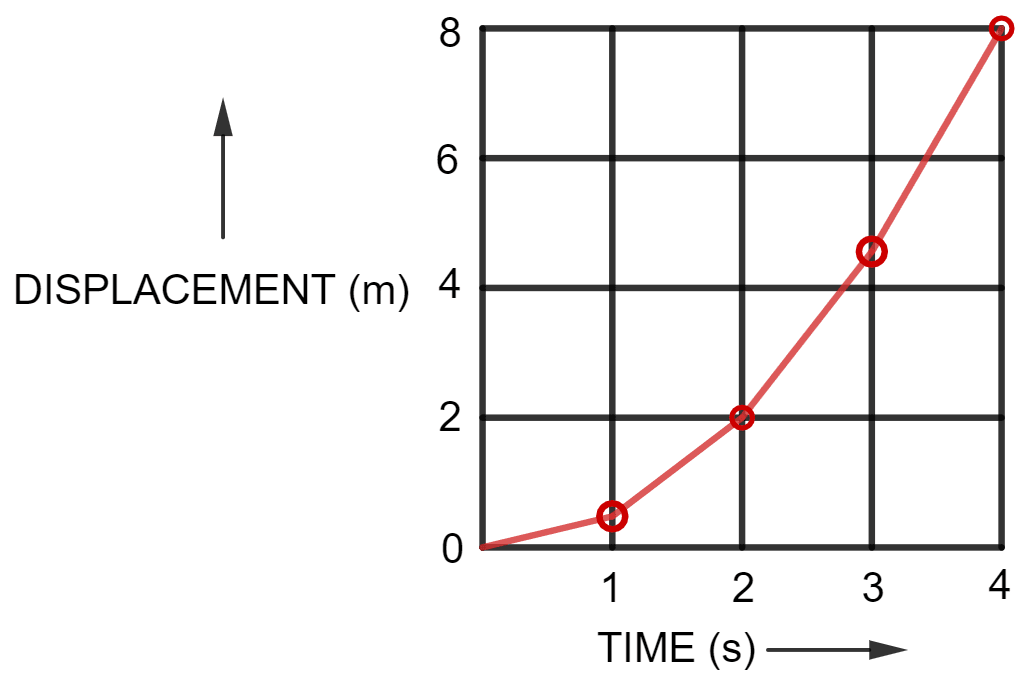#### Question 9

Figure below shows a velocity-time graph for a car starting from rest. The graph has three parts AB, BC and CD.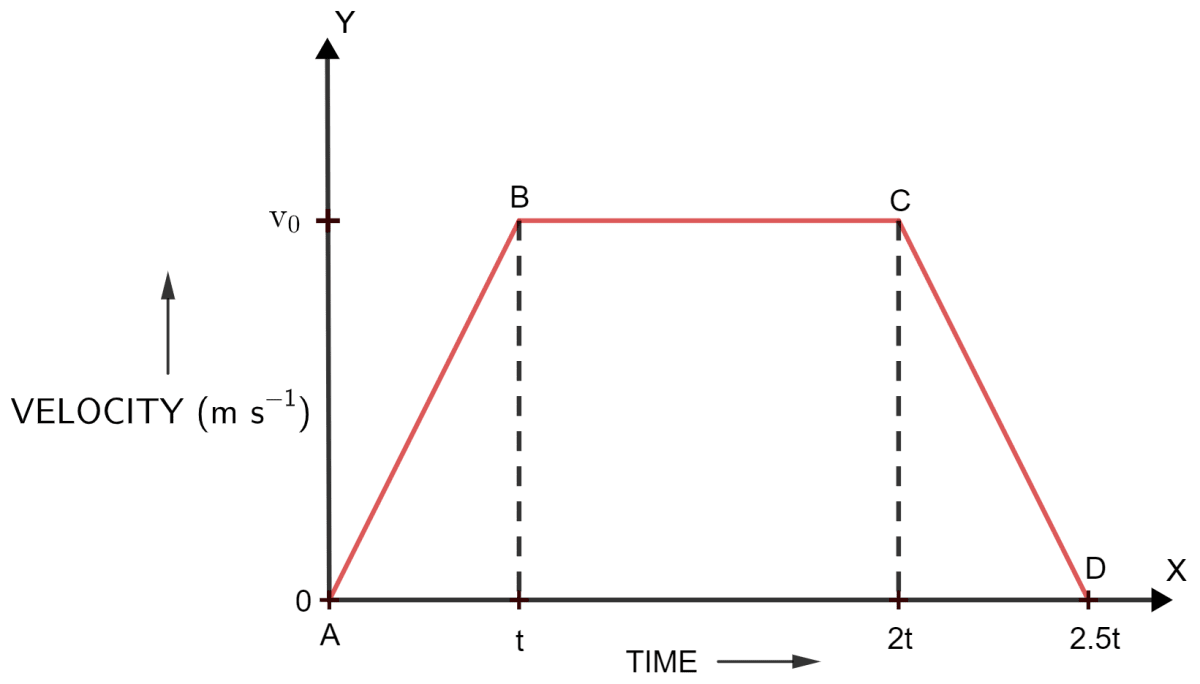(i) State how is the distance travelled in any part determined from this graph.

(ii) Compare the distance travelled in part BC with the distance travelled in part AB.

(iii) Which part of graph shows motion with uniform (a) velocity (b) acceleration (c) retardation?

(iv) (a) Is the magnitude of acceleration higher or lower than that of retardation? Give a reason. (b) Compare the magnitude of acceleration and retardation.

(i) The distance travelled in any part of the graph can be obtained by finding the area enclosed by the graph in that part with the time axis.

(ii) Let E be the point on time axis (x axis) at time t and F be the point at time 2t.

When we observe the graph, we find,

Distance travelled in part BC = Area of rectangle EBCF

∴ Distance travelled in part BC = length x breadth
= (2t - t) x (v0 - 0)
= t x v0     .... 

Distance travelled in part AB = Area of triangle ABT

∴ Distance travelled in part AB = $\dfrac{1}{2}$ x base x height
= $\dfrac{1}{2}$ x (t - 0) x (v0 - 0)

= $\dfrac{1}{2}$ x t x v0     .... 

Comparing  and  we get,

Distance travelled in part BC : Distance travelled in part AB

$= \dfrac {t v_0}{\dfrac{tv_0}{2}} \\[0.5em] = \dfrac {2}{1}$

Hence,
Distance travelled in part BC : Distance travelled in part AB = 2 : 1

(iii) The different parts of the graph are mentioned below:

(a) Uniform velocity is shown in part BC of the graph, as the velocity is constant with time.

(b) Uniform acceleration is shown in part AB of the graph, as the velocity is increasing with time.

(c) Uniform retardation is shown in part CD of the graph, as the velocity is decreasing with time.

(iv) (a) The magnitude of acceleration is lower, as slope of line AB is less than that of line CD.

(b) Acceleration in part AB = slope of AB

$\text {Slope of line AB} = \dfrac{v_0 - 0}{t - 0} \\[0.5em] \Rightarrow\text {Slope of line AB} = \dfrac{v_0}{t} \\[0.5em]$

Retardation in part CD = slope of CD

$\text {Slope of line CD} = \dfrac{v_0 - 0}{2.5t - 2t} \\[0.5em] \Rightarrow\text {Slope of line AB} = \dfrac{v_0}{0.5t} \\[0.5em]$

Magnitude of acceleration : Magnitude of retardation = Slope of line AB : Slope of line CD

$= \dfrac{\dfrac{v_0}{t}}{\dfrac{v_0}{0.5t}} \\[1em] = \dfrac{0.5}{1} \\[1em] = \dfrac{5}{10} \\[1em] = \dfrac{1}{2}$

Hence, Magnitude of acceleration : Magnitude of retardation = 1 : 2

#### Question 10

The velocity-time graph of a moving body is given below in figure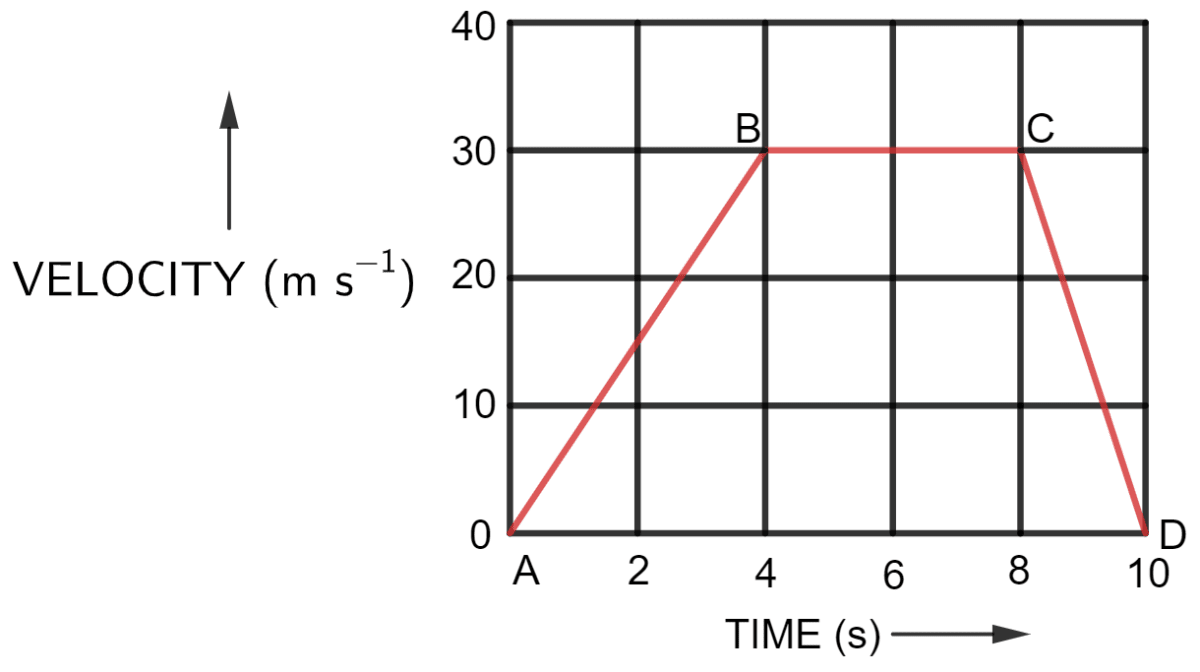Find —

(i) The acceleration in parts AB, BC and CD.

(ii) Displacement in each part AB, BC, CD, and

(iii) Total displacement.

Let E be the point at t = 4 and F be the point at t = 8 as labelled in the graph below: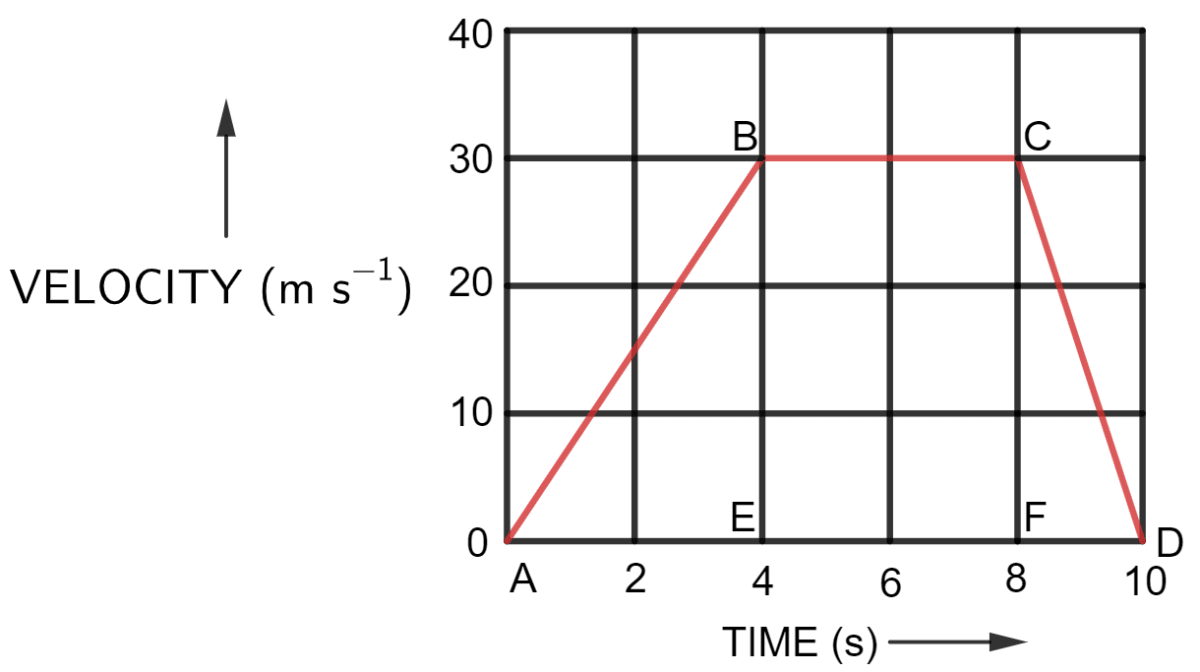(i) Acceleration in part AB = slope of AB

$\text {slope of AB} = \dfrac{(30 - 0) \text {ms}^{-1}}{(4-0) \text {s}} \\[0.5em] \text {slope of AB} = \dfrac{30 \text {ms}^{-1}}{4 \text {s}} \\[0.5em] \text {slope of AB} = 7.5 \text {ms}^{-2} \\[0.5em]$

Hence, Acceleration in part AB = 7.5 m s-2

Acceleration in part BC = slope of BC

We observe from the graph that there is no change in velocity in part BC. Hence, Acceleration in part BC = 0 m s-1

Acceleration in part CD = slope of CD

$\text {slope of CD} = \dfrac{(0 - 30) \text {ms}^{-1}}{(10-8) \text {s}} \\[0.5em] \text {slope of CD} = \dfrac{-30 \text {ms}^{-1}}{2 \text {s}} \\[0.5em] \text {slope of CD} = -15 \text {ms}^{-2} \\[0.5em]$

Hence, Acceleration in part CD = -15 m s-1

(ii) Displacement in each part is as follows —

(a) Displacement of part AB = Area of triangle ABE

$= \dfrac {1}{2} \times \text {base} \times \text {height}$

Substituting the values in the formula above, we get,

$= \dfrac {1}{2} \times {(4 -0) \text {s}} \times (30 - 0)\text {m s}^{-1} \\[0.5em] = \dfrac {1}{2} \times 4 \text {s} \times 30 \text {m s}^{-1} \\[0.5em] = 30 \text {m} \\[0.5em]$

Hence,
Displacement of part AB = 60 m

(b) Displacement of part BC = Area of Square EBCF

$= \text {length} \times \text {breadth}$

Substituting the values in the formula above, we get,

$= {(8 -4) \text {s}} \times (30 - 0)\text {m s}^{-1} \\[0.5em] = 4 \times 30 \text {m} \\[0.5em] = 120 \text {m} \\[0.5em]$

Hence,
Displacement of part BC = 120 m

(c) Displacement of part CD = Area of triangle CDF

$= \dfrac {1}{2} \times \text {base} \times \text {height}$

Substituting the values in the formula above, we get,

$= \dfrac {1}{2} \times {(10 -8) \text {s}} \times (30 - 0)\text {m s}^{-1} \\[0.5em] = \dfrac {1}{2} \times 2 \times 30 \text {m} \\[0.5em] = 30 \text {m} \\[0.5em]$

Hence,
Displacement of part CD = 30 m

(iii) Total displacement = Displacement of part AB + Displacement of part BC + Displacement of part CD
= 60 + 30 + 120 = 210

Hence,
total displacement = 210 m

#### Question 11

A ball moves on a smooth floor in a straight line with a uniform velocity 10 m s-1 for 6 s. At t = 6 s, the ball hits a wall and comes back along the same line to the starting point with same speed. Draw the velocity-time graph and use it to find the total distance travelled by the ball and its displacement.

The velocity-time graph for the motion of ball on a smooth floor in a straight line is shown below: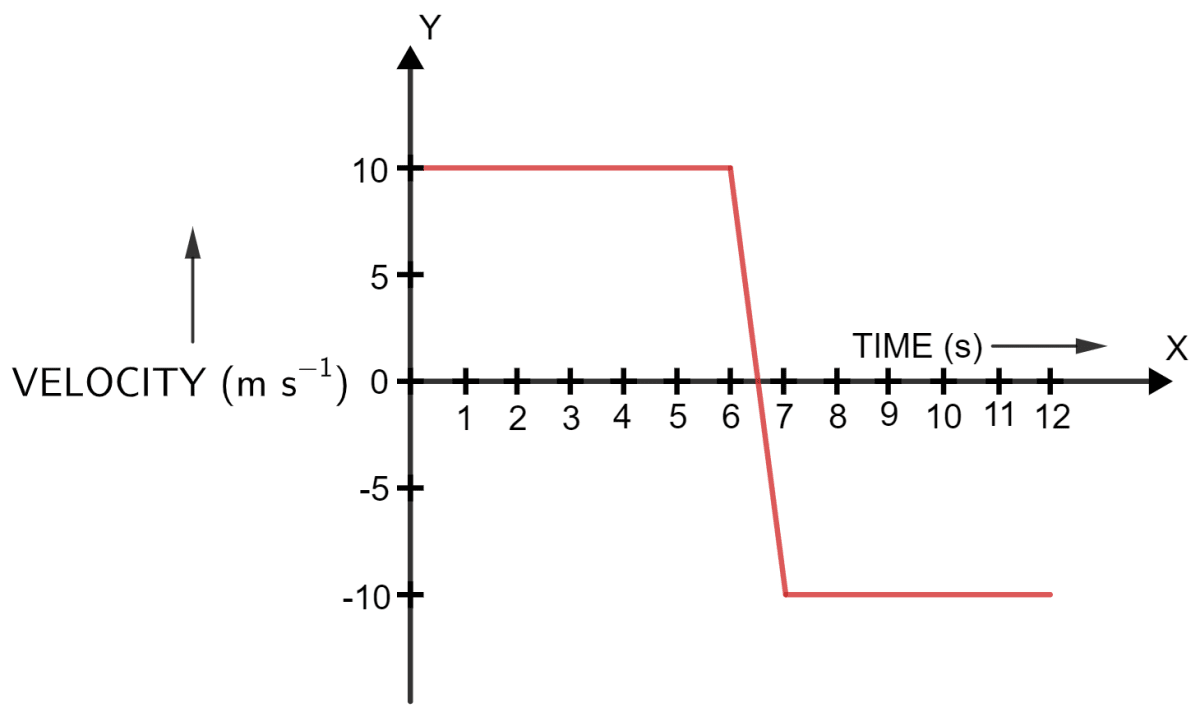Total distance travelled = distance travelled in the first 6 s + distance travelled in next 6 s.

As we know,
Distance = velocity x time

Substituting the values in the formula above we get,

Distance when the ball moves towards the wall = 10 m s-1 x 6 s = 60 m

Distance when the ball moves away from the wall = 10 m s-1 x 6 s = 60 m

Total distance = 60 m + 60 m = 120 m

Displacement = distance travelled in the forward direction - distance travelled while coming back
= 60 m - 60 m = 0

Displacement = 0 as the ball comes back to the initial place.

#### Question 12

Figure shows the velocity-time graph of a particle moving in a straight line.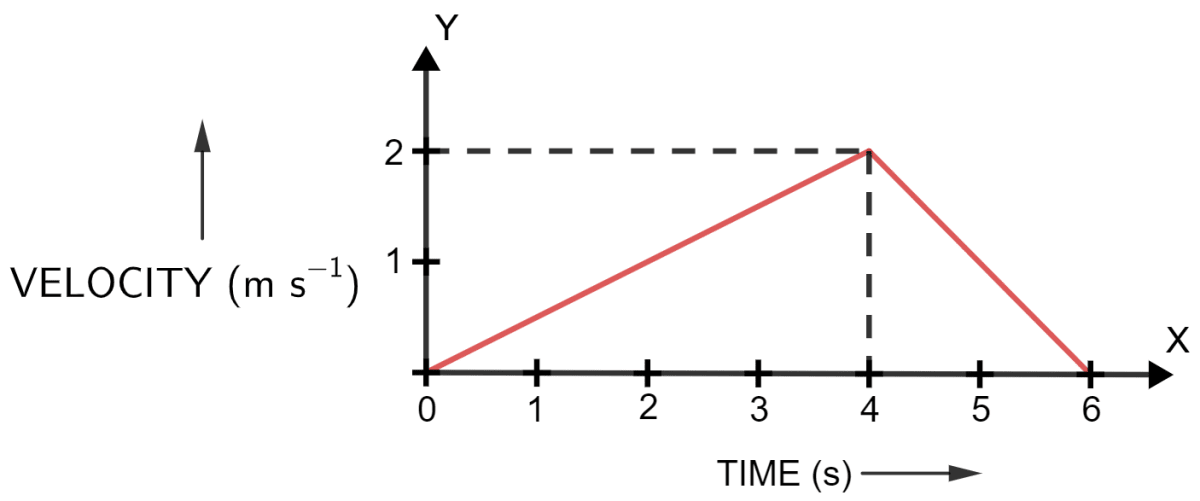(i) State the nature of motion of particle.

(ii) Find the displacement of particle at t = 6 s.

(iii) Does the particle change its direction of motion?

(iv) Compare the distance travelled by the particle from 0 to 4 s and from 4 s to 6 s.

(v) Find the acceleration from 0 to 4 s and retardation from 4 s to 6 s.

(i) As we observe the graph, we find that, the nature of motion of particle is that, the particles are uniformly accelerated from 0 to 4s and then uniformly retarded from 4s to 6s.

(ii) As we know,

displacement of particles can be obtained by finding the area enclosed by the graph in that part with the time axis up to that instance.

At t = 6 s,

Displacement = area of triangle

$= \dfrac {1}{2} \times \text {base} \times \text {height}$

Substituting the values in the formula above, we get,

$= \dfrac {1}{2} \times {(6 - 0) \text {s}} \times (2 - 0)\text {m s}^{-1} \\[0.5em] = \dfrac {1}{2} \times 6 \times 2 \text {m} \\[0.5em] = 6 \text {m} \\[0.5em]$

Hence,
Displacement of particle at t = 6 s is 6 m

(iii) No, the particle does not change its direction of motion.

(iv) At t = 0 to 4 s,

Distance covered = area of triangle

$= \dfrac {1}{2} \times \text {base} \times \text {height}$

Substituting the values in the formula above, we get,

$= \dfrac {1}{2} \times {(4 - 0) \text {s}} \times (2 - 0)\text {m s}^{-1} \\[0.5em] = \dfrac {1}{2} \times 4 \text {s} \times 2 \text {m s}^{-1} \\[0.5em] = 4 \text {m} \\[0.5em]$

Hence,
Distance covered between 0 to 4 s = 4 m

At t = 4 s to 6 s,

Distance covered = area of triangle

$= \dfrac {1}{2} \times \text {base} \times \text {height}$

Substituting the values in the formula above, we get,

$= \dfrac {1}{2} \times {(6 - 4) \text {s}} \times (2 - 0)\text {m s}^{-1} \\[0.5em] = \dfrac {1}{2} \times 2 \text {s} \times 2 \text {m s}^{-1} \\[0.5em] = 2 \text {m} \\[0.5em]$

Hence,
Distance covered between 4s to 6 s = 2 m

Distance covered between 0 to 4 s : Distance covered between 4 s to 6 s = 4 : 2 = 2 : 1

(v) Acceleration in part 0 s to 4 s = slope of graph

$\text {slope} = \dfrac{(2 - 0) \text {ms}^{-1}}{(4-0) \text {s}} \\[0.5em] \text {slope} = \dfrac{2 \text {ms}^{-1}}{4 \text {s}} \\[0.5em] \text {slope} = 0.5 \text {ms}^{-2} \\[0.5em]$

Hence,
Acceleration in part 0 s to 4 s = 0.5 m s-2

As we know,

Retardation in part 4 s to 6 s = slope of graph

$\text {slope} = \dfrac{(0 - 2) \text {ms}^{-1}}{(6 - 4) \text {s}} \\[0.5em] \text {slope} = \dfrac{- 2 \text {ms}^{-1}}{2\text {s}} \\[0.5em] \text {slope} = - 1 \text {ms}^{-2} \\[0.5em]$

Acceleration = -1 ms-2 and as we know retardation is negative acceleration

Hence, Retardation in part 4 s to 6 s = 1 m s-2

## Exercise 2(C)

#### Question 1

Write three equations of uniformly accelerated motion relating the initial velocity (u), final velocity (v), time (t), acceleration (a) and displacement (S).

The equations of uniformly accelerated motion relating to the initial velocity (u), final velocity (v), time (t), acceleration (a) and displacement (S) are as follows —

(i) v = u + at

(ii) S = ut + $\dfrac{1}{2}$ at2

(iii) v2 = u2 + 2aS

#### Question 2

Derive the following equations for a uniformly accelerated motion —

(i) v = u + at

(ii) S = ut + $\dfrac{1}{2}$ at2

(iii) v2 = u2 + 2aS

where the symbols have their usual meanings.

(i) By definition,

$\text {Acceleration} = \dfrac {\text {change in velocity}}{\text {time taken}} \\[0.5em] \Rightarrow \text {Acceleration} = \dfrac {\text {final velocity - initial velocity}}{\text {time taken}} \\[0.5em]$

Hence,

$\text {a} = \dfrac{\text{v} - \text{u}}{\text {t}} \\[0.5em] \text {at} = \text{v} - \text{u} \\[0.5em] \Rightarrow \text {v} = \text{u} + \text{at} \\[0.5em]$

Hence, we get,

v = u + at     [Eq. 1]

(ii) By definition,

$\text {Distance travelled} = \text {Avg. Velocity} \times \text{time} \\[1em] \text {Distance travelled} = \Big(\dfrac{\text {Initial Vel.} + \text {Final Vel.}}{2}\Big) \times \text{time}$

or

$\text{S} = \Big(\dfrac {\text{u} + \text{v}}{2}\Big) \times \text{t}$

But, from Eq. 1, v = u + at

Therefore,

$\text{S} = \Big(\dfrac{\text {u + ( u +at)}}{2}\Big) \times \text {t} \\[0.5em] \text{S} = \Big(\dfrac{\text {2u + at}}{2}\Big) \times \text {t} \\[0.5em] \Rightarrow \text{S} = \text {ut} + \dfrac{1}{2} \text {at}^2 \\[0.5em]$

Hence, we get,
S = ut + $\bold{\dfrac{1}{2}}$ at2

(iii) By definition,

$\text {Distance travelled} = \text {Avg. Velocity} \times \text{time} \\[0.5em] \Rightarrow \text{S} = \Big(\dfrac {\text{u} + \text{v}}{2}\Big) \times \text{t}$

But, from Eq. 1, v = u + at

or t = $\dfrac{\text{v}-\text{u}}{\text{a}}$

Therefore,

$\text{S} = \Big(\dfrac {\text{v} + \text{u}}{2}\Big) \times \Big(\dfrac{\text{v}-\text{u}}{a}\Big) \\[1em] \Rightarrow \text{S} = \dfrac{\text{v}^2 - \text{u}^2}{2\text{a}}$

So, v2 - u2 = 2aS

Hence,

v2 = u2 + 2aS

#### Question 3

Write an expression for the distance S covered in time t by a body which is initially at rest and starts moving with a constant acceleration a.

According to equation of motion —

S = ut + $\dfrac{1}{2}$ at2

Where,

S = distance covered

u = initial velocity

a = acceleration

t = time

As, body is at rest, so u = 0

Hence, we get,

$S = (0\times t) + (\dfrac{1}{2} at^2) \\[0.5em] S = 0 + (\dfrac{1}{2} at^2) \\[0.5em] \Rightarrow S = \dfrac{1}{2} at^2 \\[0.5em]$

Hence, we get,

S = $\dfrac{1}{2}$ at2

## Multiple Choice Type

#### Question 1

The correct equation of motion is —

1. v = u + 2aS
2. v = ut + a
3. S = ut + $\dfrac{1}{2}$ at2
4. v = u + at ✓

By definition,

$\text {Acceleration} = \dfrac {\text {Change in Velocity}}{\text {Time Taken}} \\[1em] \text {Acceleration} = \dfrac {\text {Final Vel. - Initial Vel.}}{\text {Time Taken}}$

Hence,

$\text {a} = \dfrac{\text{v} - \text{u}}{\text {t}} \\[0.5em] \text {at} = \text{v} - \text{u} \\[0.5em] \Rightarrow \text {v} = \text{u} + \text{at} \\[0.5em]$

Hence, we get,

v = u + at

#### Question 2

A car starting from rest accelerates uniformly to acquire a speed 20 km h-1 in 30 min. The distance travelled by car in this time interval will be —

1. 600 km
2. 5 km✓
3. 6 km
4. 10 km

As we know,

v = u + at

Given,

u = 0

v = 20 km h-1

t = 30 min = $\dfrac{1}{2}$ h

Substituting the values in the formula above, we get,

$20 = 0 + (a \times \dfrac{1}{2}) \\[0.5em] \Rightarrow a = 40 \text{ km h}^{-2} \\[0.5em]$

Now,

S = ut + $\dfrac{1}{2}$ at2

Substituting the values in the formula above we get,

$\text{S} = (0 \times 0.5) + \Big[\dfrac{1}{2} \times 40 \times \Big(\dfrac{1}{2}\Big)^2\Big] \\[0.5em] \text{S} = 0 + \Big(\dfrac{1}{2} \times 40 \times \dfrac{1}{4}\Big) \\[0.5em] \Rightarrow \text{S} = 5 \text{ km} \\[0.5em]$

Hence, distance = 5 km

## Numericals

#### Question 1

A body starts from rest with a uniform acceleration of 2 m s-2. Find the distance covered by the body in 2 s.

According to equation of motion —

S = ut + $\dfrac{1}{2}$ at2

Where,

distance covered = S

initial velocity u = 0

acceleration a = 2 m s-2

time t = 2 s

Substituting the values in the formula for u = 0 , we get,

$S = (0 \times 2) + \dfrac{1}{2} \times 2 \times 2^2 \\[0.5em] S = (0) + (\dfrac{1}{2} \times 2 \times 4) \\[0.5em] S = (0) + (4) \\[0.5em] \Rightarrow S = 4 m \\[0.5em]$

Hence, distance covered = 4 m

#### Question 2

A body starts with an initial velocity of 10 m s-1 and acceleration 5 m s-2. Find the distance covered by it in 5 s .

According to equation of motion —

S = ut + $\dfrac{1}{2}$ at2

Where,

distance covered = S

initial velocity u = 10 m s-1

acceleration a = 5 m s-2

time t = 5 s

Substituting the values in the formula, we get,

$S = (10 \times 5) + (\dfrac{1}{2} \times 5 \times 5^2) \\[0.5em] S = (50) + (\dfrac{1}{2} \times 5 \times 25) \\[0.5em] S = (50) + ( 2.5 \times 25) \\[0.5em] S = 50 + 62.5 \\[0.5em] \Rightarrow S = 112.5 m \\[0.5em]$

Hence, distance covered = 112.5 m.

#### Question 3

A vehicle is accelerating on a straight road. Its velocity at any instant is 30 km h-1, after 2 s, it is 33.6 km h-1 and after further 2 s, it is 37.2 km h-1. Find the acceleration of vehicle in m s-2. Is the acceleration uniform ?

As we know,

a = $\dfrac{v - u}{t}$

After t = 2s,

u = 30 km h-1

Converting km h-1 to m s-1

We get,

$30 \text{km h}^{-1} = \dfrac{30 \text{km}}{1\text{h}} = \dfrac {30 \times 1000 \text{m}}{60 \times 60 \text{s}} \\[0.5em] \Rightarrow 30 \text{km h}^{-1} = \dfrac {30 \times 10 \text{m}}{6 \times 6 \text{s}} \\[0.5em] \Rightarrow 30 \text{km h}^{-1} = \dfrac {8.33 \times \text{m}}{\text{s}} \\[0.5em]$

Hence, 30 km h-1 is equal to 8.33 m s-1.

Now,

v = 33.6 km h-1

Converting km h-1 to m s-1

We get,

$33.6 \text{km h}^{-1} = \dfrac{33.6 \text{km}}{1\text{h}} = \dfrac {33.6 \times 1000 \text{m}}{60 \times 60 \text{s}} \\[0.5em] \Rightarrow 33.6 \text{km h}^{-1} = \dfrac {33.6 \times 10 \text{m}}{6 \times 6 \text{s}} \\[0.5em] \Rightarrow 33.6 \text{km h}^{-1} = \dfrac {9.33 \times \text{m}}{\text{s}} \\[0.5em]$

Hence, 33.6 km h-1 is equal to 9.33 ms-1.

Substituting the values in the formula above, we get,

$a = \dfrac{9.33 - 8.33}{2} \\[0.5em] a = \dfrac{1}{2} \\[0.5em] \Rightarrow a = 0.5 m s^{2} \\[0.5em]$

Hence, acceleration in the first 2 s = 0.5 m s -2

For the next 2 s,

u = 9.33 m s-1

v = 37.2 km h-1

Converting km h-1 to m s-1

We get,

$37.2 \text{km h}^{-1} = \dfrac{37.2\text{km}}{1\text{h}} = \dfrac {37.2 \times 1000 \text{m}}{60 \times 60 \text{s}} \\[0.5em] \Rightarrow 37.2 \text{km h}^{-1} = \dfrac {37.2 \times 10 \text{m}}{6 \times 6 \text{s}} \\[0.5em] \Rightarrow 37.2 \text{km h}^{-1} = \dfrac {10.33 \times \text{m}}{\text{s}} \\[0.5em]$

Hence, 37.2 km h-1 is equal to 10.33 ms-1.

Substituting the values in the formula above, we get,

$a = \dfrac{10.33 - 9.33 }{2} \\[0.5em] a = \dfrac{1}{2} \\[0.5em] \Rightarrow a = 0.5 m s^{2} \\[0.5em]$

Hence, acceleration is 0.5 m s -2

Yes, the acceleration is uniform as the acceleration in both instances is same.

#### Question 4

A body, initially at rest, starts moving with a constant acceleration 2 m s -2.

Calculate —

(i) the velocity acquired and

(ii) and the distance travelled in 5 s.

As we know, from the equation of motion,

v = u + at

Given,

a = 2 m s -2

u = 0

t = 5 s

Substituting the values in the formula, we get,

$v = 0 + (2 ms^{-2} \times 5 s) \\[0.5em] v = 10 ms^{-1} \\[0.5em]$

Hence, the velocity acquired = 10 m s-1

(ii) According to equation of motion —

S = ut + $\dfrac{1}{2}$ at2

Substituting the values in the formula, we get,

$S = (0 \times 5) + (\dfrac{1}{2} \times 2 \times 5^2) \\[0.5em] S = 0 + (\dfrac{1}{2} \times 2 \times 5^2) \\[0.5em] \Rightarrow S = 0 + (\dfrac{1}{2} \times 2 \times 25) \\[0.5em] \Rightarrow S = 0 + 25$

Hence, distance travelled = 25 m

#### Question 5

A bullet initially moving with a velocity 20 m s-1 strikes a target and comes to rest after penetrating a distance 10 cm in the target. Calculate the retardation caused by the target.

According to equation of motion —

v2 = u2 + 2aS

Given,

u = 20 m s-1,

v = 0,

s = 10 cm = 0.1 m

Substituting the values in the formula above, we get,

$0 = (20)^2 + (2\times a \times 0.1) \\[0.5em] \Rightarrow 0 = 400 + 0.2 a \\[0.5em] \Rightarrow -400 = 0.2a \\[0.5em] \Rightarrow a = -\dfrac{400}{0.2} \\[0.5em] \Rightarrow a = -\dfrac{4000}{2} \\[0.5em] \Rightarrow a = - 2000 ms^{-2}\\[0.5em]$

Hence, Retardation is 2000 m s-2

#### Question 6

A train moving with a velocity of 20 m s-1 is brought to rest by applying brakes in 5 s. Calculate the retardation.

According to equation of motion —

v = u + at

Given,

u = 20 m s-1,

v = 0,

t = 5 s

Substituting the values in the formula above, we get,

$0 = (20) + (a \times 5) \\[0.5em] 0 = 20 + 5a \\[0.5em] \Rightarrow -20 = 5a \\[0.5em] \Rightarrow a = -\dfrac{20}{5} \\[0.5em] \Rightarrow a = - 4 ms^{-2}\\[0.5em]$

Hence, Retardation is 4 m s-2

#### Question 7

A train travels with a speed of 60 km h-1 from station A to station B and then comes back with a speed 80 km h-1 from station B to station A.

Find —

(i) the average speed, and

(ii) the average velocity of train.

As we know,

Avg Speed = $\dfrac{\text{Total Distance}}{\text{Total time}}$

Given,

From, A to B

speedAB = 60 km h-1

distance travelled = dAB = d

time taken = $\dfrac{\text{d}}{60}$

From, B to A

speedBA = 80 km h-1

distance travelled = dBA = d

time taken = $\dfrac{\text{d}}{80}$

Total distance travelled = d + d = 2d    [Eqn. 1]

Total time = $\dfrac{\text{d}}{60}$ + $\dfrac{\text{d}}{80}$    [Eqn. 2]

Substituting the values from the equations 1 and 2 in the formula we get,

$\text {Avg Speed} = \dfrac{\text {2d}}{\dfrac{\text {d}}{60} + {\dfrac{\text {d}}{80}}} \\[0.5em] \text {Avg Speed} = \dfrac{\text {2d}}{\dfrac {\text {4d + 3d}}{240}} \\[0.5em] \text {Avg Speed} = \dfrac{\text {2d}}{\dfrac {\text {7d}}{240}} \\[0.5em] \text {Avg Speed} = \dfrac{\text {2}}{\dfrac {\text {7}}{240}} \\[0.5em] \text {Avg Speed} = \dfrac {2 \times 240}{7} \\[0.5em] \text {Avg Speed} = \dfrac {480}{7} \\[0.5em] \Rightarrow \text {Avg Speed} = 68.57 \text{ km h}^{-1} \\[0.5em]$

Hence, Avg Speed = 68.57 km h-1

(ii) We know,

Average velocity = $\dfrac{\text {displacement}}{\text {total time}}$

As the train starts from station A and comes back to same station, hence, displacement is zero.

Therefore, the average velocity is also zero.

#### Question 8

A train is moving with a velocity of 90 km h-1. It is brought to stop by applying the brakes which produce a retardation of 0.5 m s-2.

Find —

(i) the velocity after 10 s, and

(ii) the time taken by the train to come to rest.

(i) As we know, according to the equation of motion,

v = u + at

Given,

u = 90 km h-1

velocity in m s-1

$90 \text{km h}^{-1} = \dfrac{90 \text{km}}{1\text{h}} = \dfrac {90 \times 1000 \text{m}}{60 \times 60 \text{s}} \\[0.5em] \Rightarrow 90 \text{km h}^{-1} = \dfrac {90 \times 10 \text{m}}{6 \times 6 \text{s}} \\[0.5em] \Rightarrow90 \text{km h}^{-1} = \dfrac {15 \times 10 \text{m}}{1 \times 6 \text{s}} \\[0.5em] \Rightarrow 90 \text{km h}^{-1} = \dfrac {25 \times \text{m}}{\text{s}} \\[0.5em]$

Hence, 90 km h-1 is equal to 25 m s-1.

v = 0 (as the train stops on application of brakes)

t = 10 s

retardation = -a = -0.5 m s-2

Substituting the values in the formula above we get,

$\text {v} = 25 + [(-0.5) \times 10] \\[0.5em] \text {v} = 25 + [- 5] \\[0.5em] \Rightarrow \text {v} = 20 \text {m s}^{-1} \\[0.5em]$

Hence, the velocity after 10 s = 20 m s-1.

(ii) As we know, according to the equation of motion,

v = u + at

Substituting the values in the formula above, to get the time taken by the train to come to rest.

$\text {0} = 25 + (-0.5 \times \text{t}) \\[0.5em] \text {0} = 25 - 0.5 \text{t} \\[0.5em] 0.5 \text{t} = 25 \\[0.5em] \Rightarrow \text {t} = \dfrac {25}{0.5} \\[0.5em] \Rightarrow \text {t} = \dfrac {250}{5} \\[0.5em] \Rightarrow \text {t} = 50 \text {s} \\[0.5em]$

Hence, the time taken by the train to come to rest = 50 s.

#### Question 9

A car travels a distance 100 m with a constant acceleration and average velocity of 20 m s-1. The final velocity acquired by the car is 25 m s-1.

Find:

(i) the initial velocity and

(ii) acceleration of car.

(i) Given,

v = 25 m s-1

As we know,

average velocity = $\dfrac{u+v}{2}$ = 20 m s-1

Substituting the value we get,

$\dfrac {u + v}{2} = 20 \\[0.5em] \dfrac{u + 25}{2} = 20 \\[0.5em] u + 25 = 20 \times 2 \\[0.5em] u + 25 = 40 \\[0.5em] \Rightarrow u = 40 - 25 = 15 m s^{-1} \\[0.5em]$

Hence, initial velocity of the car is 15 m s-1

(ii) We know, from the equation,

v2 = u2 + 2aS

S = 100 m

v = 25 m s-1

u = 15 m s-1

Substituting the values in the formula above we get,

${25} ^2 = {15}^2 + (2 \times a \times 100) \\[0.5em] 625 = 225 + (200 \times a) \\[0.5em] 625 - 225 = (200 \times a) \\[0.5em] 400 = (200 \times a) \\[0.5em] \Rightarrow a = \dfrac {400}{200} \\[0.5em] \Rightarrow a = 2 \text{m s}^{-2}\\[0.5em]$

Hence, acceleration of car = 2 m s-2

#### Question 10

When brakes are applied to a bus, the retardation produced is 25 cm s-2 and the bus takes 20 s to stop.

Calculate —

(i) the initial velocity of bus, and

(ii) the distance travelled by bus during this time.

As we know,

v = u + at

Given,

Retardation = -a = -25 cm s-2

Expressing it in m s-2

25 cm s-2 = $\dfrac{25}{100}$m s-2

Hence, -a = -0.25 m s-2

t = 20 s

v = 0

u = ?

Substituting the values in the formula above we get,

$0 = u + (- 0.25) \times 20 \\[0.5em] 0 = u - 5.0 \\[0.5em] \Rightarrow u = 5 \text{ms}^{-1}$

Hence, the initial velocity of bus = 5 m s-1

(ii) As we know, from the equation of motion,

v2 = u2 + 2aS

Substituting the values, we get,

$0^2 = 5^2 + [2 \times (- 0.25) \times S] \\[0.5em] 0 = 25 - [0.50 \times S] \\[0.5em] 0.50 \times S = 25 \\[0.5em] \Rightarrow S = \dfrac{25}{0.5} \\[0.5em] \Rightarrow S = \dfrac{250}{5} \\[0.5em] \Rightarrow S = 50 m \\[0.5em]$

Hence, distance travelled = 50 m

#### Question 11

A body moves from rest with a uniform acceleration and travels 270 m in 3 s. Find the velocity of the body at 10 s after the start.

As we know,

S = ut + $\dfrac{1}{2}$ at2

Given,

u = 0 m s -1

S = 270 m

t = 3 s

Substituting the values in the formula above we get,

$270 = (0 \times 3) + (\dfrac{1}{2} \times a \times 3^2) \\[0.5em] 270 = \dfrac{9}{2} \times a \\[0.5em] 270 \times 2 = 9 \times a \\[0.5em] \Rightarrow a = \dfrac{540}{9} \\[0.5em] \Rightarrow a = 60 \text{ms}^{-2}$

Hence, a = 60 m s-2

To find out velocity after t = 10 s.

v = u + at

Substituting the values in the formula above we get,

$v = 0 + (60 \times 10) \\[0.5em] \Rightarrow v = 600 \text{ms}^{-1} \\[0.5em]$

Hence, velocity after 10 s = 600 m s-1

#### Question 12

A body moving with a constant acceleration travels the distances 3 m and 8 m respectively in 1 s and 2 s.

Calculate —

(i) the initial velocity, and

(ii) the acceleration of body.

As we know,

S = ut + $\dfrac{1}{2}$at2

Let,

S1 = 3 m

t1 = 1 s

S2 = 8 m

t2 = 2 s

Substituting the values in the formula above we get,

$3 = (u \times 1) + (\dfrac{1}{2} \times a \times 1^2) \\[0.5em] 3 = u + (\dfrac{1}{2}a) \\[0.5em] 3 = u + \dfrac{a}{2} \\[0.5em] 2 \times (3 - u) = a \\[0.5em] \Rightarrow a = 6 - 2u \qquad \bold{....[Eqn. \space 1]}$

For 8m distance,

$8 = (u \times 2) + (\dfrac{1}{2} \times a \times 2^2) \\[0.5em] 8 = 2u + (\dfrac{1}{2} \times 4a) \\[0.5em] 8 - 2u = 2a \\[0.5em] 2 \times (4 - u) = 2a \\[0.5em] \Rightarrow a = 4 - u \qquad \bold{....[Eqn. \space 2]}$

Solving Equations 1 & 2,

a = 6 - 2u      [Eqn. 1]

a = 4 - u        [Eqn. 2]

we get,

0 = 2 - u
⇒ u = 2

Hence, initial velocity = 2 m s -1

Putting the value of u in Equation 2 and solving for a:

$a = 4 - u \\[0.5em] \Rightarrow a = 4 - 2 \\[0.5em] \Rightarrow a = 2 m s ^{-2}\\[0.5em]$

Hence, the acceleration of body is 2 m s-2

#### Question 13

A car travels with a uniform velocity of 25 m s-1 for 5 s. The brakes are then applied and the car is uniformly retarded and comes to rest in further 10 s.

Find —

(i) the distance which the car travels before the brakes are applied,

(ii) the retardation and

(iii) the distance travelled by the car after applying the brakes.

(i) As we know,

Distance = Speed x time

Initial velocity = u = 25 m s-1

Final velocity = 0

time = 5 s

Substituting the values in the formula above we get,

$\text{Distance} = 25 \times 5 \\[0.5em] \Rightarrow \text{Distance} = 125 m$

Hence, distance covered = 125 m.

(ii) Retardation = -a = $\dfrac{v - u}{t}$

t = 10 s

Substituting the values in the formula above we get,

$\text {Retardation} = -\dfrac{0 - 25}{10} \\[0.5em] \Rightarrow \text {Retardation} = \dfrac{25}{10} \\[0.5em] \Rightarrow \text {Retardation} = 2.5 \text {m s}^{-2} \\[0.5em]$

Hence, retardation of the car = 2.5 m s-2

(iii) As we know,

v2 - u2 = 2aS

Substituting the values in the formula above we get,

$(0)^2 - (25)^2 = 2 \times (-2.5) \times S \\[0.5em] 0 - 625 = - 5S \\[0.5em] \Rightarrow - 5S = - 625\ \\[0.5em] \Rightarrow S = \dfrac{625}{5} \\ \\[0.5em] \Rightarrow S = 125 m \\ \\[0.5em]$

Hence, the distance travelled by the car after applying the brakes = 125 m.

#### Question 14

A space craft flying in a straight course with a velocity of 75 km s-1 fires its rocket motors for 6.0 s. At the end of this time, its speed is 120 km s-1 in the same direction.

Find —

(i) the space craft’s average acceleration while the motors were firing

(ii) the distance travelled by the space craft in the first 10 s after the rocket motors were started, the motors having been in action for only 6.0 s.

(i) As we know,

v - u = at

Given,

u = 75 km s-1

v = 120 km s-1

t = 6 s

Substituting the values in the formula above we get,

$120 - 75 = a \times 6 \\[0.5em] 45 = 6a \\[0.5em] \Rightarrow a = \dfrac{45}{6} \\[0.5em] \Rightarrow a = 7.5 \text { km s}^{-2} \\[0.5em]$

Hence, acceleration = 7.5 km s-2

(ii) As we know,

S = ut + $\dfrac{1}{2}$at2

For the first 6 s,

u = 75 km s-1

a = 7.5 km s-2

$S_{1} = (75 \times 6) + (\dfrac{1}{2} \times 7.5 \times 6^2) \\[0.5em] S_{1} = 450 + (\dfrac{1}{2} \times 7.5 \times 36) \\[0.5em] S_{1} = 450 + (7.5 \times 18) \\[0.5em] S_{1} = 450 + 135 \\[0.5em] \Rightarrow S_{1} = 585 \text { km}$

Hence, S1 = 585 km

For the next 4 s

S2 = speed x time

Given,

t = 4 s

speed = 120 km s -1

Substituting the values in the formula above, we get,

$S_2 = 120 \times 4 \\[0.5em] S_2 = 480 \text { km}$

Hence, S2 = 480 km

Total distance covered by the aircraft = S1 + S2
= 585 + 480
= 1065 km

Hence,
Total distance covered by the aircraft = 1065 km.

#### Question 15

A train starts from rest and accelerates uniformly at a rate of 2 m s-2 for 10 s. It then maintains a constant speed for 200 s. The brakes are then applied and the train is uniformly retarded and comes to rest in 50 s.

Find —

(i) the maximum velocity reached,

(ii) the retardation in the last 50 s,

(iii) the total distance travelled, and

(iv)the average velocity of the train.

As we know,

v = u + at

Given,

u = 0

t = 10 s

a = 2 m s-2

Substituting the values in the formula above we get,

$v = 0 + 2 \times 10 \\[0.5em] \Rightarrow v = 20 \\[0.5em]$

Hence, final velocity = 20 m s-1

(ii) Retardation in last 50 s,

As we know,

v = u + at

Given,

u = 20 m s-1

v = 0 m s-1

t = 50 s

Substituting the values in the formula above we get,

$0 = 20 + [(- a) \times (50)] \\[0.5em] \Rightarrow 50 \times a = 20 \\[0.5em] \Rightarrow a = \dfrac{20}{50} \\[0.5em] \Rightarrow a = 0.4 \text{ m s}^{-2} \\[0.5em]$

Hence, retardation in the last 50 s = 0.4 m s-2

(iii) As we know,

S = ut + $\dfrac{1}{2}$ at2

For the first 10 s,

u = 0

a = 2 ms-2

$S_{1} = (0 \times 10) + (\dfrac{1}{2} \times 2 \times 10^2) \\[0.5em] S_{1} = 0 + (\dfrac{1}{2} \times 2 \times 100) \\[0.5em] \Rightarrow S_{1} = 100 \text{ m}$

Hence, S1 = 100 m   .... 

For the next 200 s,

S2 = speed x time

where speed = 20

time = 200 s

Substituting the values in the formula above we get,

$S_2 = 20 \times 200 \\[0.5em] \Rightarrow S_2 = 4000 \text{ m} \\[0.5em]$

Hence, S2 = 4000 m   .... 

For the next 50 s

$S_{3} = (20 \times 50) + [\dfrac{1}{2} \times (- 0.4) \times 50^2] \\[0.5em] S_{3} = 1000 - [0.2 \times 2500] \\[0.5em] S_{3} = 1000 - [0.2 \times 2500] \\[0.5em] \Rightarrow S_{2} = 1000 - 500 \text{ m} \\[0.5em] \Rightarrow S_{2} = 500 \text{ m}$

Hence, S3 = 500 m   .... 

So,

Total distance = sum of ,  and 

$S = 100 \text{ m} + 4000 \text{ m} + 500 \text{ m} \\[0.5em] \Rightarrow S = 4600 \text{ m}$

Hence, total distance covered = 4600 m

(iv) average velocity = $\dfrac{\text {total distance covered}}{\text {time taken}}$

Substituting the values in the formula above we get,

$\text {average velocity} = \dfrac{4600}{260} \\[0.5em] \Rightarrow \text {average velocity} = 17.69 \text{ m s}^{-1}$

Hence, average velocity = 17.69 m s-1